# 1 Overview

This report presents results from application of the Data File Orientation Toolkit to your data file. It includes a number of insights about your data file that provide valuable information for using your data for research.

The toolkit begins by guiding qualitative assessment of the dataset’s relevance. This is a static part of the report that is important for assessing the data file’s usefulness for research. The main sections focus on three sets of quantitative analyses for your data file: (1) accuracy of the data values, (2) completeness of the records in the dataset, and (3) comparability of the data values among groups and over time. Analyses in this report are informed by recommended practices from the data quality literature and are selected for their importance for common kinds of research questions.

The findings of this report will help the user understand the strengths and weaknesses of their data file for conducting research. We include a series of analyses with discussion of how to interpret each and what further steps may be taken when a possible issue is detected. Results may inform decisions regarding how to use the data file for research as well as any caveats to draw from research findings. Further, findings may inform feedback for future maintenance of the data file.

This report is particularly geared toward state and local administrative datasets and includes guidance for the issues common to these datasets. While the design of this report is tailored to such datasets, other data files with similar formats may also benefit from the use of the toolkit.

When a strange result or pattern is detected in these analyses, it is often difficult to know whether the pattern reflects an issue with the data or just a notable pattern, for example changes in benefit amounts due to legal changes. While the toolkit will often not be able to distinguish between data issues and other notable patterns, observing these patterns can be highly informative for understanding your data and guiding interpretations of research findings. Further, understanding the program details affecting the dataset analyzed can greatly assist with interpretation of findings in this report.

Among the many analyses available in this report, the user may navigate to the sections of most interest using the links available in the Table of Contents above.

This report is generated using R Markdown, available with R and RStudio. The sections of this report include R code chunks that refer to different code needed to implement analyses. Convenient defaults are included for this code for the example data file ‘test_data_file.csv’ provided. We encourage the user to modify the script to the specific needs of their analyses. For each section, the R script to refer to for making changes is designated by the “Reference Script.”

Places where we recommend the user consider modifying the code are designated in the report below and in the README file on our GitHub repo. We also have set up the R code to read inputs from modifying “setup.yml.” Thus, a user can make modifications to this input file for key inputs for the toolkit in place of modifying the R code.

For troubleshooting relating to output for this report, please see the instructions in the README on our GitHub page and refer to R documentation for more specific issues.

# 2 Format of Analyses for the Report

In the analyses that follow, you will see results from different analyses regarding your data. Each set of analyses is organized as follows:

1. Description
1. Describes the question(s) the analysis seeks to answer about the file.
2. Describes the analyses (graphs, tables, other) that will be applied to help answer the question(s).
2. Code and Output
1. A “code chunk” is provided that demonstrates the R code used to run the analysis on the dataset. While the code includes convenient defaults for analyses of the example file provided, guidance is also provided for how a user may adapt aspects of the analysis to better fit their needs.
2. Usually a table or figure is provided as output from the analysis.
3. A description of how to read the table or figure is provided.
3. Interpretation
1. Discusses what kinds of patterns a user should look for in the data and the possible meaning of different patterns.
2. Relates the output to the initial questions for the analysis. Suggests next steps for further investigation.

These analyses are meant to provide a better understanding of your data, its strengths and weaknesses, and any areas of caution needing further attention or care when conducting research.

# 3 Analysis Preview

In the analyses that follow, the toolkit begins by guiding a qualitative assessment of Relevance. Then, the main sections of the toolkit cover three primary components to understand your data file - Accuracy, Completeness, and Comparability.

## 3.1 Relevance

Relevance of the data derives from an analysis of metadata and documentation and is a crucial first step of data quality analysis. Some factors include indicators such as the suitability of the source in terms of the statistical population, units, variables, reference time, and domain variables.

## 3.2 Accuracy

In the context of this report, accuracy entails the data file’s conformity to expectations of values. Each column should have a standard set or range of values that each entry can follow. However, inaccurate data might contain values that break this expected conformity. For example, in a column that has entries for zip codes, inaccuracies may take the form of non-valid zip codes or invalid data formats.

## 3.3 Completeness

Completeness examines such questions as whether data cover the population of interest, include correct records, and do not contain duplicate or out-of-scope records. (A check for duplicates is planned for a later version of the toolkit.) Additionally, completeness includes whether cases have information filled in for all appropriate fields without missing data.

Note that accuracy and completeness analyses are included in the same section of this report, as some analyses address both dimensions.

## 3.4 Comparability

Comparability is the extent to which differences among statistics reflect real phenomena rather than methodological differences. Types of comparability include over time, across geographies, and among domains.

Different analyses in this (or other) section may be more or less important based on the user’s research questions. For example, if the user is particularly interested in making comparisons in outcomes among groups, comparability analyses among groups may be particularly important.

# 4 Relevance

An important first step for analyzing a data file’s utility for a research question is to assess the dataset in terms of its relevance, or suitability in terms of such components as the population, units, and variables. In particular, a review of the metadata and documentation available is valuable for assessing the dataset’s relevance, in addition to contacting the agency maintaining the data for specific questions. Complete and reliable metadata can be critical toward assessing the suitability of the dataset as well as possible limitations of the analysis. These assessments are conducted before proceeding with the other steps, to avoid spending time and resources on examining other data quality aspects for a data source that is not relevant.

In addition to reviewing metadata and documentation, it is also important to understand how legal changes and changes in eligibility for program receipt may affect the suitability of the data source for research. We recommend the user gather available information on the legal and programmatic requirements that may affect the data source.

The following list of questions can guide you in the assessment of the relevance of the data file as well as the identification of limitations that will affect the interpretations of the results of the analysis. It is adapted from Laitila et al. (2011).

Category Question Description/Example
1. Units 1.1. What unit is represented at the record level of the data file? For example, persons, households, transactions.
1.2. Do grouped units represent another aggregate unit? For example, households as a group of persons
1.3. Are the units included in the data file relevant to the study? In many cases, the unit of the administrative data is different from the relevant unit of analysis. Often, administrative data is at the event level (for example, payment to a benefit recipient). A dataset that only includes information at the recipient level may not be relevant for such an analysis.
2. Population 2.1. Is the population of units in the data file what is needed for the study? Administrative data stores detailed information of a particular group that is of interest for the administration of the activity or program. However, this group may or may not be the relevant population for analysis.
3. Variables 3.1. What variables in the data capture conceptually what is of interest for the research? For example, whether an income variable in the dataset captures the correct income concept of interest for research.
4. Time 4.1. Is the time reference of the data set what is needed for the analysis? For example, to study the effect of a new policy, data will need to be available from after the policy was implemented.
4.2. Is there administrative delay in the data? In some cases data are recorded sometime after an event occurs. For example, the employment status of a benefit recipient could change in practice, but this might not be reflected until the next time a benefit program’s data file is updated with information from the employment records system.
5. Groups 5.1. What groups of interest are needed in the analysis? E.g. race, poverty, location.
5.2. What variables can help to identify these relevant groups? Are there variables to distinguish different groups of interest for comparison? These are also called domain variables.
6. Policy changes 6.1 What changes in policy and in law occurred during the reference time period? 6.2 Do any of these changes impact your analysis? For example, when there are changes to the tax code, the data collected on tax forms may also change and may impact time series estimates constructed from income tax records.

# 5 Data File Input

Reference Script: Toolkit.Rmd

NOTE: Any edits to the code in this section can be made to Toolkit.Rmd. For subsequent sections, the reference script to edit is indicated.

Once the dataset is judged to be relevant for the analysis, it might require some processing before continuing to the next steps. The following steps will guide you to define the relevant parameters of the toolkit.

The initially provided code is based on the example data file included on the GitHub repo ‘test_data_file.csv’, representing longitudinal benefits data for a benefits program. A user may input a flat file in a similar format for analysis with the toolkit, with requirements described the README on the GitHub site.

Defining file name

``````##### EDIT THIS SECTION TO DEFINE FILE NAME #####

### Read in data file for toolkit analyses

Creating variables

This section reads in parameters needed for the different variables to be analyzed from the setup file setup.yml. Please refer to the README and setup file for instructions for setup.yml.

``````# THIS SECTION READS FROM THE FILE SETUP.YML WHICH INCLUDES ALL THE VARIABLE DESCRIPTIONS
all_variables <- names(input_yaml\$variables)
variables_dataframe <- lapply(input_yaml\$variables, data.frame)
variables_dataframe_filled <- rbind.fill(variables_dataframe)
variables_dataframe_filled['name'] <- all_variables

#####################################################``````

Defining relevant variables

In this section, the user should define the relevant variables for the analysis.

NOTE: All inputs here are set up automatically for users who use the setup.yml input file.

At least one ID, time, key, and domain variable are required, which should be indicated in the id_vars, time_vars, key_vars, and domain_vars sets below. If there are no time variables, then using a filler variable with the same value for all records, for example ‘1’ can be used. The user can optionally specify location variables in location_vars.

Then, the user should identify all the character variables in charvars set below, which are all variables who are neither numeric nor dates, including IDs. It is important to make sure that only variables that were identified in the previous step are included here. Finally, the character variables are converted into factors and the user should indicate the labels of these variables where appropriate.

NOTE: There is a section below indicated “EDIT THIS SECTION TO DEFINE LABELS OF CHARACTER VARIABLES” where the user may apply labels for any categorical variables in the dataset. Some examples are provided for two variables from the example data file.

``````# Setting relevant variables as defined by yaml. At least one variable for each of the following 4 groups should be included.
id_vars <- variables_dataframe_filled[variables_dataframe_filled\$classification=='id', 'name']
time_vars <- variables_dataframe_filled[variables_dataframe_filled\$classification=='time', 'name']
key_vars <- variables_dataframe_filled[variables_dataframe_filled\$classification=='key', 'name']
domain_vars <- variables_dataframe_filled[variables_dataframe_filled\$classification=='domain', 'name']

# Location variables can optionally be specified
location_vars <- variables_dataframe_filled[variables_dataframe_filled\$classification=='location', 'name']

# We only keep variables selected by the user
analysis_file <- select(analysis_file,c(id_vars, time_vars, location_vars, key_vars, domain_vars))

# Modifying categorical variables to be strings and factors
to_categories <- variables_dataframe_filled[variables_dataframe_filled\$type=='categorical', 'name']
analysis_file <- analysis_file[, (to_categories):=lapply(.SD, as.factor), .SDcols = to_categories]
analysis_file <- analysis_file[, (to_categories):=lapply(.SD, as.character), .SDcols = to_categories]

##### EDIT THIS SECTION TO DEFINE LABELS OF CHARACTER VARIABLES  #####
analysis_file\$source <- factor(analysis_file\$source, levels=c(1,2) , labels = c("FundSource1","FundSource2"))
analysis_file\$other_benef <- factor(analysis_file\$other_benef, levels=c(1,2) ,  labels = c("Yes", "No"))

######################################################################

# The rest of the variables will be defined as numeric and data is converted to data frame
charvars <- variables_dataframe_filled[variables_dataframe_filled\$type=='character', 'name']
numvars <- variables_dataframe_filled[variables_dataframe_filled\$type=='numeric', 'name']
analysis_file <- as.data.frame(analysis_file)
analysis_file[,numvars] <- sapply(analysis_file[,numvars],as.numeric)
str(analysis_file)``````

Defining subsetting parameters

In this section, the user should specify the parameters to subset the data file, if needed, using a regular expression in quotation marks (""). The default applies no subsetting, which is indicated by two quotation marks with no characters in between.

``````##### SELECT SUBSETTING PARAMETERS #####
# This example subsets file to records prior to July 2015
subset_param <- ""
########################################

# Subsetting dataset
subset_text <- subset_param
if (subset_param =="" | is.null(subset_param)) {
subset_param <- TRUE
subset_text <- "All"
}

# If error in subsetting parameters, we use all the data
subsetted <- try( subset(analysis_file,eval(parse(text = paste0(subset_param)))), silent=TRUE)
if (class(subsetted) == "try-error") {
print("Subsetting parameters are not correct. Using all the data.")
subset_text <- "All"
subsetted <- analysis_file
}

analysis_file <- subsetted``````

# 6 Accuracy and Completeness

## 6.1 Do the data conform to expected rules and value ranges?

Reference Script: DataChecks\Data_Checks.Rmd

Description: To perform data analysis it is important to understand the quality of the inputs to the analysis. This section compares the variables of interest to their respective formatting rules. If, for example, a variable called “county” based on FIPS codes may only assume integer values between 1 and 999 then any value above or below these thresholds or any non-integer value would be indicated as of dubious quality. A record which does not conform to this rule could imply that the variable for that specific record is inaccurate or in extreme cases that the entire record is of suspect quality. Ideally, the review of any deviations from expected formats should be performed by an analyst with a solid understanding of the nature of the data and what the deviations may mean for analysis that will use the indicated records. Here we use various visualizations to explore the quality and amount of format deviations for each variable of interest, as well as the dataset overall, and finally at the record-level.

### 6.1.1 Checks for variable rules and value ranges (based on codebook)

In this section begin by defining any variables that will be useful for determining codebook adherence of the dataset. If a codebook is not available, this section can be used to check rules that the variables would be expected to adhere to. For example, a regular expression and evaluation of that expression for each value in the data can be used to create a binary “pass/fail” variable to use in the codebook validation.

Notes for Setup: In the below code, all expressions for rules to check are placed within the validator function. Each argument to the validator function has three components: (1) a new variable which has the same name as the variable of interest concatenated with the suffix “c_”, (2) the variable of interest, and (3) the rule that the variable of interest should follow (e.g. a range of values, certain length of characters). Care should be taken to ensure that the rule matches the intended specifications and that the rule will be specified appropriately given the variable’s class.

``````##### SELECT VARIABLES FOR DESCRIPTIVE STATISTICS OUTPUT#####
selected_var <- c(key_vars,domain_vars,id_vars,time_vars,location_vars)

##### EDIT THIS SECTION TO CREATE ADDITIONAL VARIABLES FOR QUALITY ASSESSMENT AND TO DEFINE CODEBOOK RULES #####

#zip_str <- "((^[0-9]{5}\$)|(^[0-9]{4}\$)|(^[0-9]{3}\$)|(^[0-9]{5}-[0-9]{4}\$)|(^[0-9]{5}-\$)|(^[0-9]{4}-\$)|(^[0-9]{3}-\$))"
#analysis_file\$zip_detect <- str_detect(analysis_file\$zip, zip_str)
#analysis_file\$zip_detect[analysis_file\$zip_detect==TRUE] <- "Plausible"
#analysis_file\$zip_detect[analysis_file\$zip_detect==FALSE] <- "Suspect"

v <- validator(
c_month = as.numeric(month) >= 200101 & as.numeric(month) <=201805 & as.numeric(month) %% 1 ==0 & as.numeric(substr(as.character(200101),5,6)) %in% c(1:12) ,
c_hh_id = nchar(trim(hh_id)) >= 1,
#  c_zip = zip_detect=="Plausible",
c_source = source %in% c('FundSource1', 'FundSource2'),
c_other_benef = other_benef %in% c("Yes", "No"),
c_amount = amount>=0,
c_type_case = type_case %in% c('Type1', 'Type2', 'Type3'),
c_num_recip = num_recip %in% c(0:30)
)

##############################################################################

cf <- confront(analysis_file[,c(selected_var)],v)
#cf <- confront(analysis_file[,c(selected_var,"zip_detect")],v)
summcf <- summary(cf)

summcf\$fail_perc <- with(summcf,100*fails/(items-nNA))

# THIS CODE ASSUMES THAT THE NAMES USED IN THE VALIDATOR RULES PREFIX EACH VARIABLE NAME WITH 2 CHARACTERS (E.G. "c_")
summcf\$variable <- substring(summcf\$name,3,str_length(summcf\$name))

#print(summcf[,1:7])
kable(summcf[,c(10,2:(ncol(summcf)-1))],caption="Rule Checks by Variable")``````
Rule Checks by Variable
variable items passes fails nNA error warning expression fail_perc
month 897717 897717 0 0 FALSE FALSE as.numeric(month) >= 200101 & as.numeric(month) <= 201805 & as.numeric(month)%%1 == 0 & as.numeric(substr(as.character(200101), 5, 6)) %vin% c(1:12) 0
hh_id 897717 897717 0 0 FALSE FALSE nchar(trim(hh_id)) >= 1 0
source 897717 857717 0 40000 FALSE FALSE source %vin% c(“FundSource1”, “FundSource2”) 0
other_benef 897717 897717 0 0 FALSE FALSE other_benef %vin% c(“Yes”, “No”) 0
amount 897717 857717 0 40000 FALSE FALSE (amount - 0) >= -1e-08 0
type_case 897717 897717 0 0 FALSE FALSE type_case %vin% c(“Type1”, “Type2”, “Type3”) 0
num_recip 897717 897717 0 0 FALSE FALSE num_recip %vin% c(0:30) 0

The summcf object includes metrics regarding the quality of each variable in the data

“Name” is the rule/variable name

“Items” is the total number of records considered

“Passes” is the total number of records that follow the rule

“Fails” is the total number of records that violate the rule

“nNA” is the total number of records that have a missing/NA value

“Error” indicates that the rule is not validly specified and should be corrected (e.g. variable name incorrect or rule is syntactically incorrect)

“Warning” indicates that the rule generates a warning and may require further consideration

“Expression” is the rule/expression that was evaluated

“Fail_perc” is the percentage of non-null values that do not adhere to the codebook rules

What to look for:

• Number of fails: A high number of fails indicates a variable of poor data quality. Consideration should be given to this variable if it will be used in any analysis.

• Number of missing values: A high number of missing (nNA) values indicates potentially incomplete data. Consideration should be given to this variable if it will be used in any analysis.

• Error in the expression: If the “Error” field equals “TRUE” then the rule for the variable is misspecified. The rule should be evaluated for accuracy and appropriateness given the class of the variable.

• Warning in the expression: If the “Warning” field equals “TRUE” then the rule for the variable may have an issue. The rule should be evaluated for accuracy and appropriateness given the class of the variable.

### 6.1.2 Visualization of variable-level rule checks

Here we visualize the distribution of accuracy/completeness for each key variable chosen. The data quality is then analyzed by a grouping variable. Variables that do not deviate from codebook formatting guidelines will not be displayed.

``````summcf_incna<- summcf[which(summcf\$fails>0 | summcf\$nNA>0),]

if (nrow(summcf_incna)==0) {
cat("All of the records adhere to the codebook rules for all values.  There is no more output to display")
} else {
plot1 <-  barplot(100*t(prop.table(as.matrix(summcf_incna[,3:5]),1)),
legend = c("Correct","Incorrect","Missing"),
args.legend = list(x='top',cex=.7),
col=c("blue","orange","gray"),
cex.names=.7,
axisnames=T,
names.arg=summcf_incna\$variable,
las=1,
axes=T,
space=1,
xlab="Percent",
width=1,
ylim=c(0,(3*nrow(summcf_incna))),
horiz=T,
main=paste("Distribution of codebook conformity by variable"))
text(0,plot1,sprintf("%2.1f",signif(100*t(prop.table(as.matrix(summcf_incna[,3:5]),1))[1,],3)),col="white",cex=.8,pos=4)
cat("All other fields comply with codebook and do not have missing values.")
}``````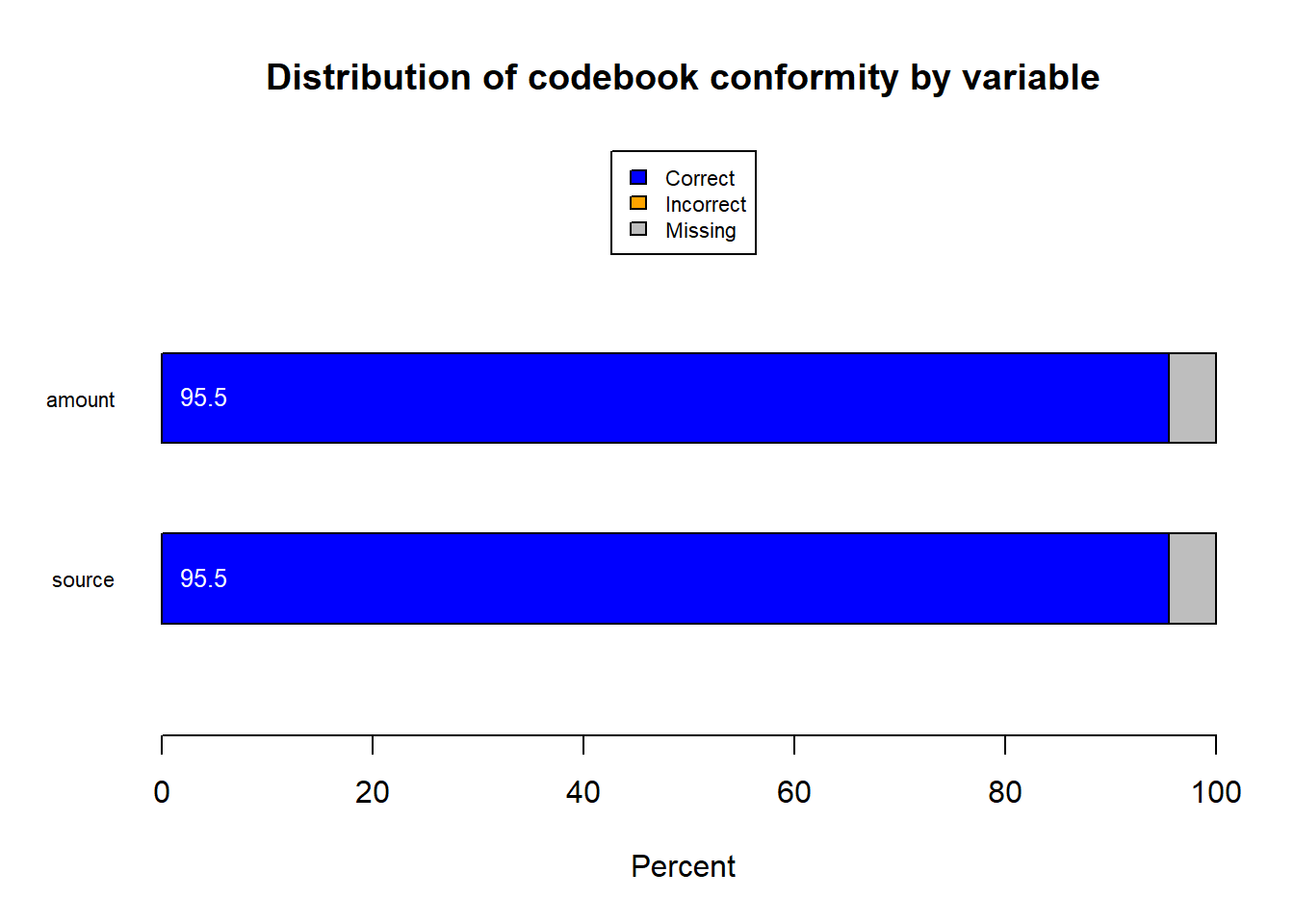``## All other fields comply with codebook and do not have missing values.``

Interpretation: Variables with higher levels of fails and missingness may require further consideration before using them in an analysis. For example, missing values may be imputed when a model can be constructed to accurately estimate their values.

When missingness is related to key variables for analysis, excluding the records with missing values from the analysis can lead to systematic errors. Imputation of missing data is one common way to resolve this issue. For resources on imputation, see Schafer (1997) or Van Buuren (2018).

### 6.1.3 Overall fail metrics across all variables

``````if (nrow(summcf_incna)>0) {
items <- sum(summcf\$items)
fails <- sum(summcf\$fails)
nNA <- sum(summcf\$nNA)

# OVERALL FAIL METRICS ACROSS ALL VARIABLES
overall_fail_perc <- 100*fails/(items-nNA)
fail_perc_min <- min(summcf\$fail_perc,na.rm=TRUE)
fail_perc_max <- max(summcf\$fail_perc,na.rm=TRUE)
fail_perc_med <- median(summcf\$fail_perc,na.rm=TRUE)
any_fail <- subset(summcf,fail_perc > 0,select=variable)
any_fail <- as.character(any_fail)
any_fail <- gsub(pattern="c\\(",replacement="", x=any_fail)
any_fail <- gsub(pattern="\\)",replacement="", x=any_fail)
any_fail <- gsub(pattern="\"",replacement="", x=any_fail)

# BECAUSE R WILL DEFAULT TO STORING MANY SIGNIFICANT DIGITS FOR THE PERCENTAGES AND BECAUSE IT MAY OR MAY NOT STORE THEM IN SCIENTIFIC NOTATION FORMAT, IT IS NECESSARY TO FORCE THE FORMATTING TO BE IN SCIENTIFIC NOTATION TO MAKE AUTOMATED CODING POSSIBLE. THE FOLLOWING CODE ENSURES THAT ALL VALUES ARE CONSISTENTLY STORED IN SCIENTIFIC NOTATION.

fail_perc_max <- format(fail_perc_max, scientific=T)
scaleplot <- as.numeric(substring(fail_perc_max,regexpr("e",fail_perc_max)+2,str_length(fail_perc_max)))

scaleplot <- as.numeric(substring(fail_perc_max,regexpr("e",fail_perc_max)+2,str_length(fail_perc_max)))

cat(paste("The overall fail rate is",
formatC(overall_fail_perc, format = "e", digits = 2)))
cat("\n")
cat(paste("The lowest fail rate for any variable is",formatC(fail_perc_min, format = "e", digits = 2)))
cat("\n")
cat(paste("The highest fail rate for any variable is",formatC(fail_perc_max, format = "e", digits = 2)))
cat("\n")
cat(paste("The median fail rate across variables is",formatC(fail_perc_med, format = "e", digits = 2)))
cat("\n")
cat(paste("The following variables had at least one failure:",as.character(any_fail)))
}``````
``````## The overall fail rate is 0.00e+00
## The lowest fail rate for any variable is 0.00e+00
## The highest fail rate for any variable is 0e+00
## The median fail rate across variables is 0.00e+00
## The following variables had at least one failure: character(0``````

Interpretation: Understanding to what degree the data adhere to the codebook will help determine what analyses are appropriate. The overall, lowest, highest, and median fail rates all give an indication of the quality of the data. Variables with at least one failure can be investigated further to better understand the degree to which data quality may be compromised. In some cases the reasons for having inconsistent values in the data can be adequately understood and the values can be recoded. In other cases the data may be best left as is in order to avoid introducing inaccuracies.

## 6.2 How are the data distributed? Are there any values which seem atypical or implausible when compared to the overall distribution of the data?

Reference Script: distribution_analysis\outliers.rmd

Description: It is always advisable to understand the distributional characteristics of the data that one is working with prior to investigating associative relationships and conducting other types of analysis. Understanding which variables are qualitative, categorical, ordinal, and continuous, for example, allows the user to create appropriate specifications in models. Additionally, knowing how the data are distributed gives the user a sense of the range of values that are likely for a given variable. Sometimes this will suggest the application of some form of scaling to make the data more suitable for analysis. It may also help uncover the presence of outliers which will require special handling. Furthermore, sometimes variables are of limited interest when they only assume a few values since the lack of variation does not differentiate the records in meaningful ways. The user is encouraged to discover other reasons for why the distributional characteristics of the data are important for their specific use case.

### 6.2.1 Descriptive Statistics for Numeric Variables

``````##### EDIT THIS SECTION TO DEFINE THE LOCATION TO STORE THE DESCRIPTIVE STATISTICS AND TO SELECT THE APPROPRIATE DATA FOR ANALYSIS#####

##### SELECT VARIABLES FOR DESCRIPTIVE STATISTICS OUTPUT#####
selected_var <- c(key_vars, domain_vars)
############################
########################################################

# BASIC DATA QUALITY
num.file <- "dq_num.csv"
cat.file <- "dq_cat.csv"
checkDataQuality(data= analysis_file[,selected_var], out.file.num= num.file, out.file.cat= cat.file)``````
``````## Check for numeric variables completed // Results saved to disk // Time difference of 1.73778 secs
## Check for categorical variables completed // Results saved to disk // Time difference of 2.592642 secs``````
``````### Numeric Variables: Data Quality Check

kable(numfile,caption="Numeric Variable Descriptive Statistics")``````
Numeric Variable Descriptive Statistics
X non.missing missing missing.percent unique mean min p1 p5 p10 p25 p50 p75 p90 p95 p99 max
amount 857717 40000 4.46 1995 368.18 20 62 138 175 235 326 456 539 599 769 5000
num_recip 897717 0 0.00 7 2.24 1 1 1 1 1 2 3 4 4 6 7

Interpretation: Evaluate the descriptive statistics for each numeric variable and determine if the values and ranges are reasonable. Explore anomalous results in more detail.

### 6.2.2 Descriptive Statistics for Character Variables

``````### Character Variables: Data Quality Check

kable(charfile,caption="Character variables descriptives")``````
Character variables descriptives
X n.non.miss n.miss n.miss.percent n.unique cat_1 freq_1 cat_2 freq_2 cat_3 freq_3
source 857717 40000 4.46 3 FundSource1 841370 FundSource2 16347 NA
other_benef 897717 0 0.00 2 Yes 781988 No 115729 NA
type_case 897717 0 0.00 3 Type2 440820 Type1 434005 Type3 22892

Interpretation: Evaluate the descriptive statistics for each character variable and determine if the unique values and frequency for each are appropriate. Explore anomalous results in more detail.

### 6.2.3 Distributions, Extreme Values, and Outliers

For variables that are continuous, it is appropriate and important to explore the distributional quality of the data. The identification of skewness, deviation from normality, and outliers can be included in the list of important steps in the data evaluation process. Traditional boxplots which use four quantiles (i.e. quartiles) can be helpful in understanding basic distributional characteristics (e.g. median, interquartile range) from certain types of data. However, they are limited in their ability to differentiate between extreme values and outliers, and do not provide as granular distribution information as other methods especially in the tail of the distribution where it is often desirable to understand more.

One method that satisfies these needs and can supplement the boxplot is the letter value plot. The letter value plot dynamically determines the appropriate number of quantiles to use to best fit the data. Use multiple analytical methods including boxplots, letter value plots, outlier detection and descriptive statistics to gain a more complete picture of the data and to inform the interpretation of analysis.

Interpretation of Boxplots and Letter Value Plots

Boxplots divide the ordered data into four groups such that the 25% of the data representing the lowest values are grouped into the first quartile. The second quartile is composed of the next 25% lowest values and so on. From this grouping the distribution is realized with the median represented by the middle bar that divides the two boxes. The whiskers represent the range of the more extreme values of the data. And the individual data points, if there are any, represent data that may be outliers. Outliers are determined according to the method of “fences” as outlined by Tukey (1977). In this method the interquartile range is multiplied by either 1.5 or 3 and the result is added or subtracted from the appropriate quartile. Points that fall beyond these thresholds are identified as potential outliers.

The letter value plot approach divides the data into a determined number of quantiles as opposed to the automatic four in the boxplot. The exact number of quantiles (boxes) that are created depends on the number of records, the distributional quality of the data, and the level of uncertainty in the quantiles’ upper and lower bounds. Similarly, the identification of outliers (data points) is performed in an adaptive way, flagging records that fall outside of the most extreme quantiles. For more details on letter value plots and the determination of outliers see Hofmann, Wickham, and Kafadar (2017).

``````##### EDIT THIS SECTION TO DEFINE CONTINUOUS VARIABLES OF INTEREST#####
contvars <- as.character(numfile\$X)

########################################################

testnums <- contvars
rm_vars <- as.character()
for (i in 1:length(testnums)) {
min <- min(analysis_file[,testnums[i]],na.rm = T)
max <- max(analysis_file[,testnums[i]],na.rm = T)
if (min==max) print(paste0("Variable ", testnums[i], " assumes only 1 value across the entire dataset. That value is ",min,". Plots and outlier status will be suppressed."))
if (min==max) rm_vars=rbind(rm_vars,testnums[i])
}
contvars=setdiff(contvars,rm_vars)

for (i in c(1:length(contvars))) {
cat("\n")
cat("\n","Distributions, Plots and Potential Outliers for",contvars[i])
if (length(unique(analysis_file[[contvars[i]]])) < 30) {
tablecont <-kable(table(analysis_file[[contvars[i]]],useNA="ifany",dnn=c(contvars[i])))
print(tablecont)
} else {
print(histogram(analysis_file[[contvars[i]]],xlab=contvars[i]))
}
desc <- summary(analysis_file[[contvars[i]]])
iqr <- desc["3rd Qu."] - desc["1st Qu."]

cat("\n")
print(kable(table(analysis_file[[contvars[i]]]> desc["3rd Qu."]+1.5*iqr,dnn="Mild High Outlier")))
maxout<- analysis_file[which(analysis_file[[contvars[i]]]> desc["3rd Qu."]+1.5*iqr),]
print(paste("Number of mild high outliers based on quartile method for variable",contvars[i],"is",nrow(maxout)))
print(kable(table(analysis_file[[contvars[i]]]> desc["3rd Qu."]+3*iqr,dnn="Extreme High Outlier")))
maxout<- analysis_file[which(analysis_file[[contvars[i]]]> desc["3rd Qu."]+3*iqr),]
print(paste("Number of extreme high outliers based on quartile method for variable",contvars[i],"is",nrow(maxout)))

print(kable(table(analysis_file[[contvars[i]]]< desc["1st Qu."]-1.5*iqr,dnn="Mild Low Outlier")))
minout <- analysis_file[which(analysis_file[[contvars[i]]]< desc["1st Qu."]-1.5*iqr),]
print(paste("Number of mild low outliers based on quartile method for variable",contvars[i],"is",nrow(minout)))
print(kable(table(analysis_file[[contvars[i]]]< desc["1st Qu."]-3*iqr,dnn="Extreme Low Outlier")))
minout <- analysis_file[which(analysis_file[[contvars[i]]]< desc["1st Qu."]-3*iqr),]
print(paste("Number of extreme low outliers based on quartile method for variable",contvars[i],"is",nrow(minout)))

boxplot(analysis_file[[contvars[i]]],main=paste("Boxplot for",contvars[i],"variable"))

LVboxplot(analysis_file[!is.na(analysis_file[[contvars[i]]]),contvars[i]], alpha = 0.95, k = NULL, perc = NULL,
horizontal = TRUE, xlab = contvars[i], ylab = NULL, col = "grey30",
bg = "grey90", width = 0.9, width.method = "linear",
median.col = "grey10",
main=paste("Letter Value Plot for",contvars[i],"variable"))

lv_table <- lvtable(analysis_file[!is.na(analysis_file[[contvars[i]]]),contvars[i]],k=determineDepth(nrow(analysis_file[!is.na(analysis_file[[contvars[i]]]),]),alpha=.05),alpha=.95)

lv_table<-cbind(lv_table,rep(0,nrow(lv_table)))
colnames(lv_table)<-"lv_thresh_h"
lv_table<-cbind(lv_table,rep(0,nrow(lv_table)))
colnames(lv_table)<-"lv_thresh_l"
lv_table=as.data.frame(lv_table)

med <- which(row.names(lv_table)=="M")

# HIGH OUTLIER
for (j in c((med+1):(nrow(lv_table)-1))) {
lv_table[j,'lv_thresh_h']<-  ifelse(lv_table[j,'97.5%']>lv_table[j+1,'2.5%'] ,1,0)
}
# LOW OUTLIER
for (k in c((med-1):2)) {
lv_table[k,'lv_thresh_l']<-  ifelse(lv_table[k,'2.5%']<lv_table[k-1,'97.5%'] ,1,0)
}

lv_table[is.na(lv_table[['lv_thresh_h']]),'lv_thresh_h'] <-  0
lv_table[is.na(lv_table[['lv_thresh_l']]),'lv_thresh_l'] <-  0

if (any(lv_table[['lv_thresh_h']]==1)) {
lv_threshval_h<-min(subset(lv_table,lv_thresh_h==1,select="97.5%"))
print(kable(table(analysis_file[[contvars[i]]]>=lv_threshval_h,dnn="Letter Value High Outliers")))
print(paste0("Number of letter value high outliers for variable ",contvars[i]," is ",nrow(subset(analysis_file,get(contvars[i])>=lv_threshval_h)),".  Values above ",lv_threshval_h," are considered outliers with this method."))
}  else {
print(paste("There are no letter value high outliers for variable",contvars[i]))
}

if (any(lv_table[['lv_thresh_l']]==1)) {
lv_threshval_l<-max(subset(lv_table,lv_thresh_l==1,select="2.5%"))
print(kable(table(analysis_file[[contvars[i]]]<=lv_threshval_l,dnn="Letter Value Low Outliers")))
print(paste0("Number of letter value low outliers for variable ",contvars[i]," is ",nrow(subset(analysis_file,get(contvars[i])<=lv_threshval_l)),".  Values below ",lv_threshval_l," are considered outliers with this method."))
}  else {
print(paste("There are no letter value low outliers for variable",contvars[i]))
}
}``````
``````##
##
##  Distributions, Plots and Potential Outliers for amount``````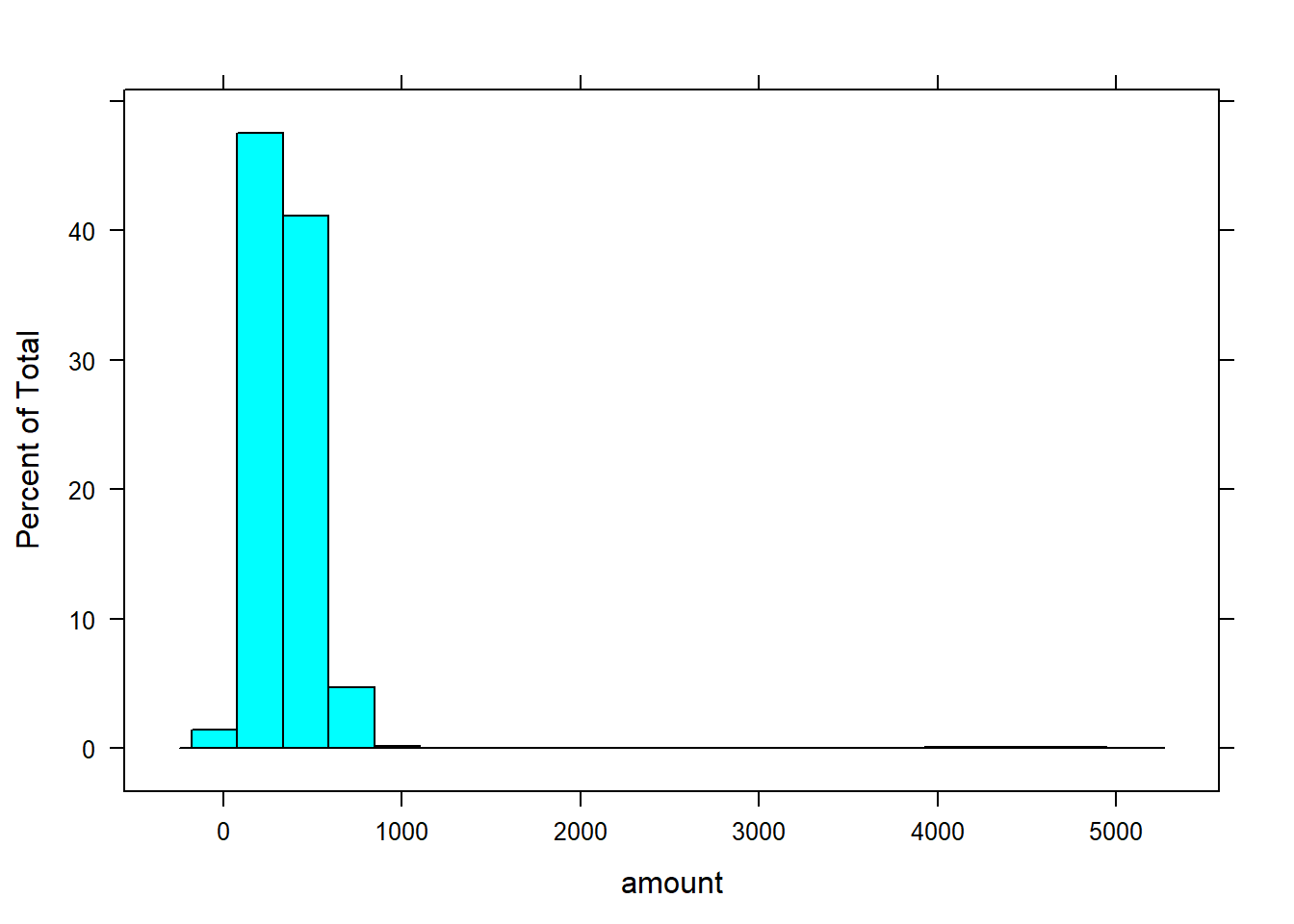``````##
##
##
## Mild.High.Outlier      Freq
## ------------------  -------
## FALSE                850007
## TRUE                   7710
##  "Number of mild high outliers based on quartile method for variable amount is 7710"
##
##
## Extreme.High.Outlier      Freq
## ---------------------  -------
## FALSE                   853156
## TRUE                      4561
##  "Number of extreme high outliers based on quartile method for variable amount is 4561"
##
##
## Mild.Low.Outlier      Freq
## -----------------  -------
## FALSE               857717
##  "Number of mild low outliers based on quartile method for variable amount is 0"
##
##
## Extreme.Low.Outlier      Freq
## --------------------  -------
## FALSE                  857717
##  "Number of extreme low outliers based on quartile method for variable amount is 0"``````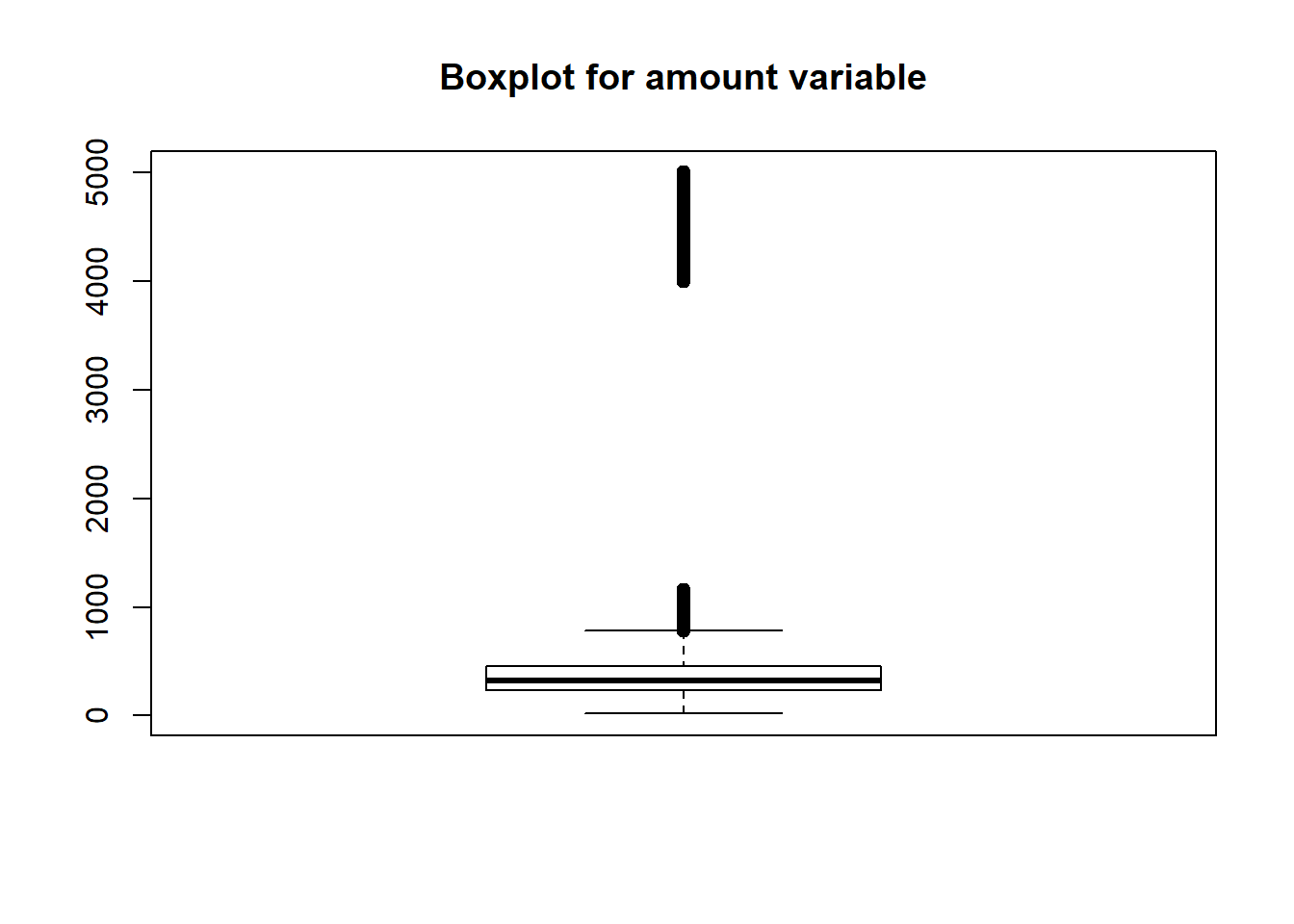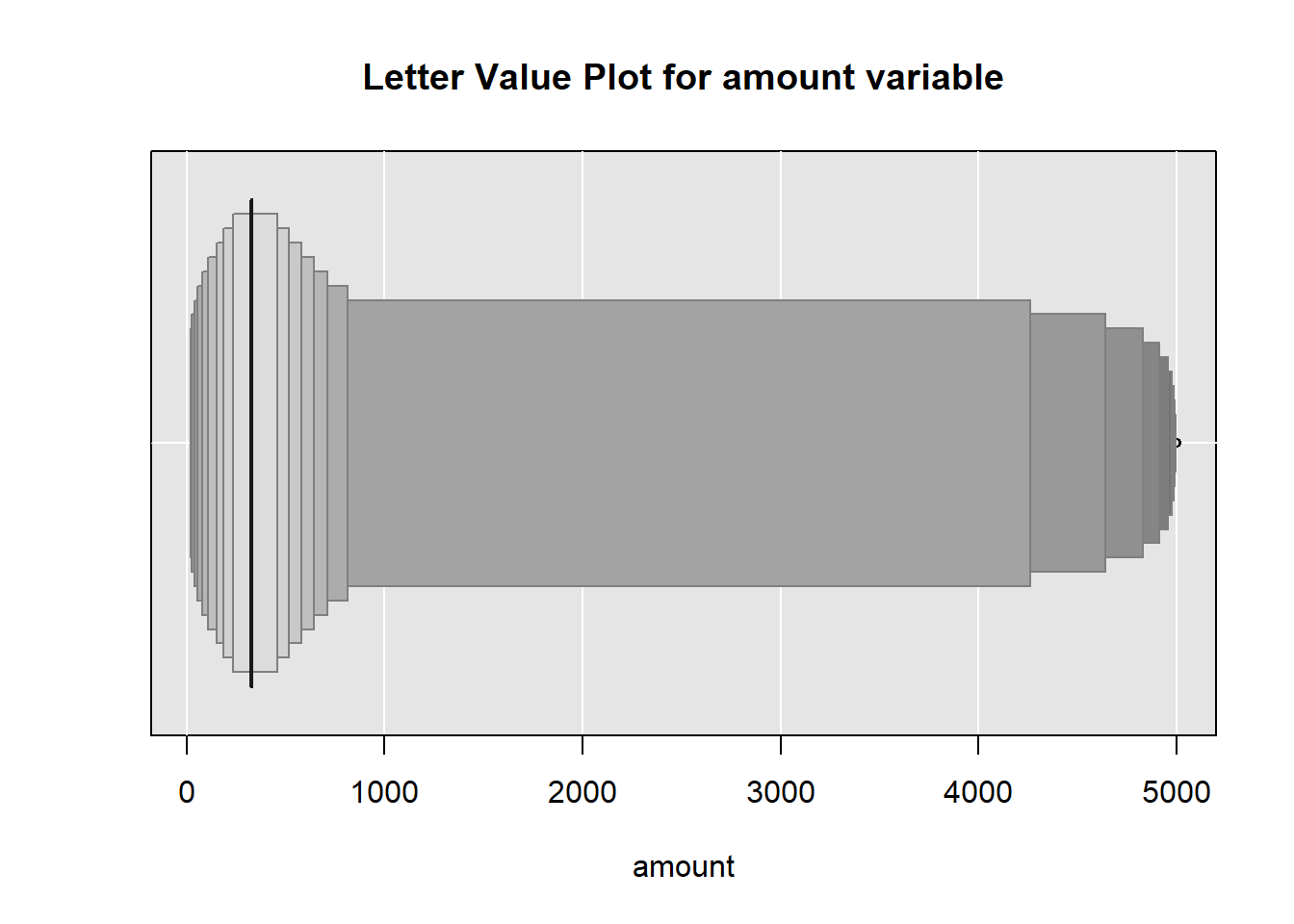``````##
##
## Letter.Value.High.Outliers      Freq
## ---------------------------  -------
## FALSE                         857697
## TRUE                              20
##  "Number of letter value high outliers for variable amount is 20.  Values above 4994 are considered outliers with this method."
##  "There are no letter value low outliers for variable amount"
##
##
##  Distributions, Plots and Potential Outliers for num_recip
##
## num_recip      Freq
## ----------  -------
## 1            256623
## 2            350522
## 3            169155
## 4             77682
## 5             29072
## 6              9762
## 7              4901
##
##
##
## Mild.High.Outlier      Freq
## ------------------  -------
## FALSE                892816
## TRUE                   4901
##  "Number of mild high outliers based on quartile method for variable num_recip is 4901"
##
##
## Extreme.High.Outlier      Freq
## ---------------------  -------
## FALSE                   897717
##  "Number of extreme high outliers based on quartile method for variable num_recip is 0"
##
##
## Mild.Low.Outlier      Freq
## -----------------  -------
## FALSE               897717
##  "Number of mild low outliers based on quartile method for variable num_recip is 0"
##
##
## Extreme.Low.Outlier      Freq
## --------------------  -------
## FALSE                  897717
##  "Number of extreme low outliers based on quartile method for variable num_recip is 0"``````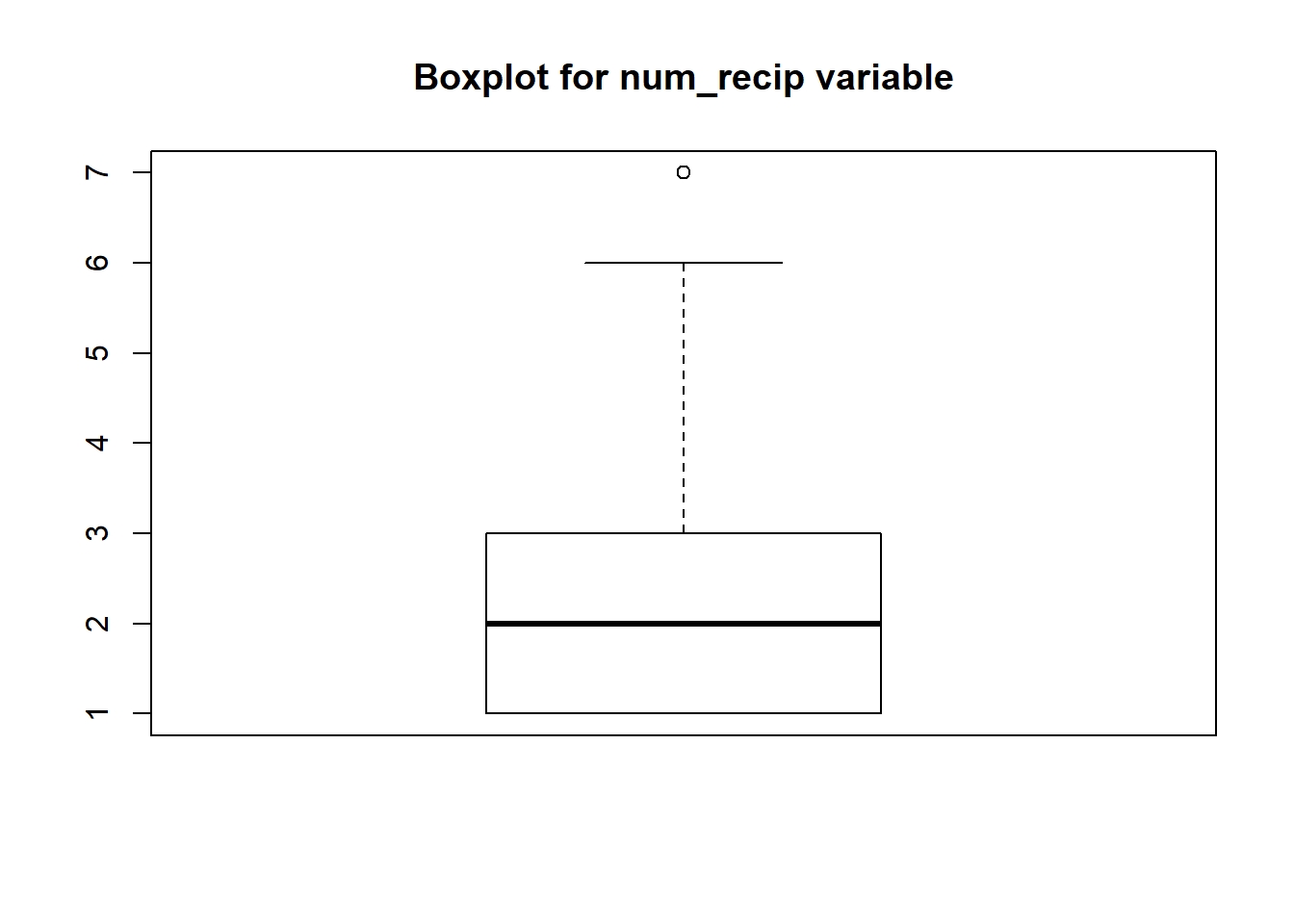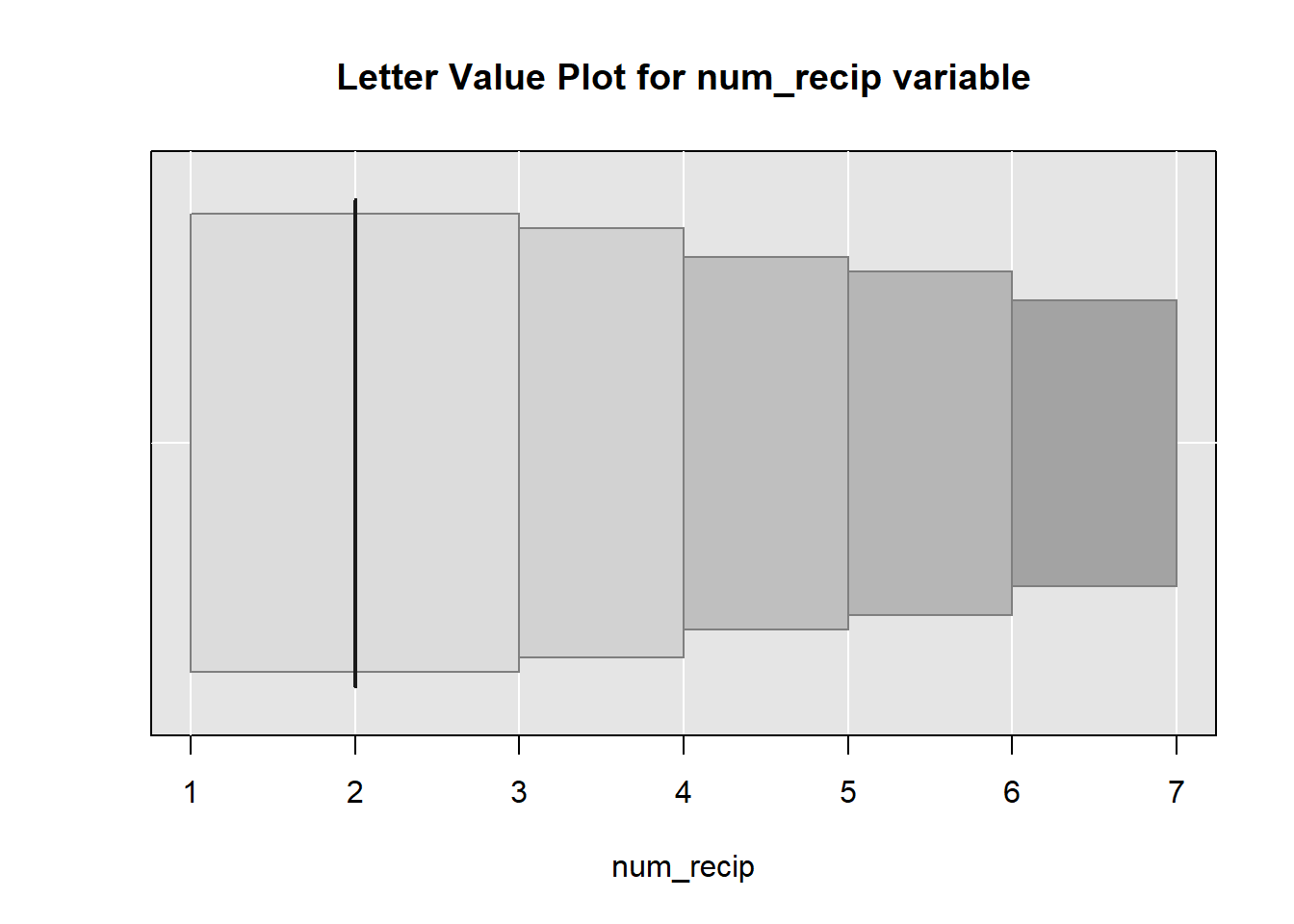``````##  "There are no letter value high outliers for variable num_recip"
##  "There are no letter value low outliers for variable num_recip"``````

Interpretation: The text output bring attention to variables which potentially outliers for various threshold settings. It also identifies variables that assume only one value in across the entire dataset and therefore would be of little interest for analysis. The boxplots and letter value plots help visualize the overall distribution while bringing attention to the potential outliers.

When outliers are identified it may indicate possible data quality issues. It is suggested that the user research these data points in further detail to determine the accuracy of these values. The inclusion of these data in analytical processes such as regression modeling may not necessarily be appropriate and could lead to incorrect conclusions. The user is advised to consider these issues when performing data analysis.

## 6.3 How do variable distributions compare to expected distributions?

Reference Script: distribution_analysis\examine_var_distributions.Rmd

Description: Beyond outlier detection, this set of analyses examines the distribution of key and domain variables regarding incongruences with expected distributions. In many cases, judgment will be needed to determine if there is a problem in the distribution, although some guidance can be provided to look for particular features of relevance. For example, in the case of a variable measuring income, it is expected that the distribution will be skewed to the right, with few observations with very high values.

We use histograms to assess the distribution of continuous variables and frequency bar plots for categorical or factor variables.

### 6.3.1 Distributions of key and domain variables

We graph the distribution of the key and domain variables. In the case of histograms, a line is also graphed that indicates the median value of the distribution.

Histograms and frequency bar plots: For each key and domain variable, we provide either a histogram or a frequency bar plot to assess the distribution.

``````# Remove the use of scientific notation
options(scipen = 999)

# Histograms and frequencies of all key and domain variables
for (i in c(key_vars, domain_vars)) {

if (class(subsetted[[i]]) == "integer" | class(subsetted[[i]]) == "numeric" | class(subsetted[[i]]) == "double") {
suppressMessages(print(ggplot(subsetted, aes_string(x=i)) +
geom_histogram() +
theme_economist() +
ggtitle(paste0("Histogram of ", i), subtitle = paste0("Subset: ",subset_text,". Median value in blue." )) +
geom_vline(aes_string(xintercept=median(subsetted[[i]],na.rm=TRUE)), color="blue", linetype="dashed", size=1)))
}

else if (class(subsetted[[i]]) == "factor" | class(subsetted[[i]]) == "character") {
suppressMessages(print(ggplot(data=subsetted, aes_string(x = i)) +
geom_bar() +
theme_economist() +
scale_x_discrete(labels = function(x) str_wrap(x, width = 10)) +
ggtitle(paste0("Frequency of ", i), subtitle = paste0("Subset: ",subset_text )) ) )
}

}``````
``## Warning: Removed 40000 rows containing non-finite values (stat_bin).``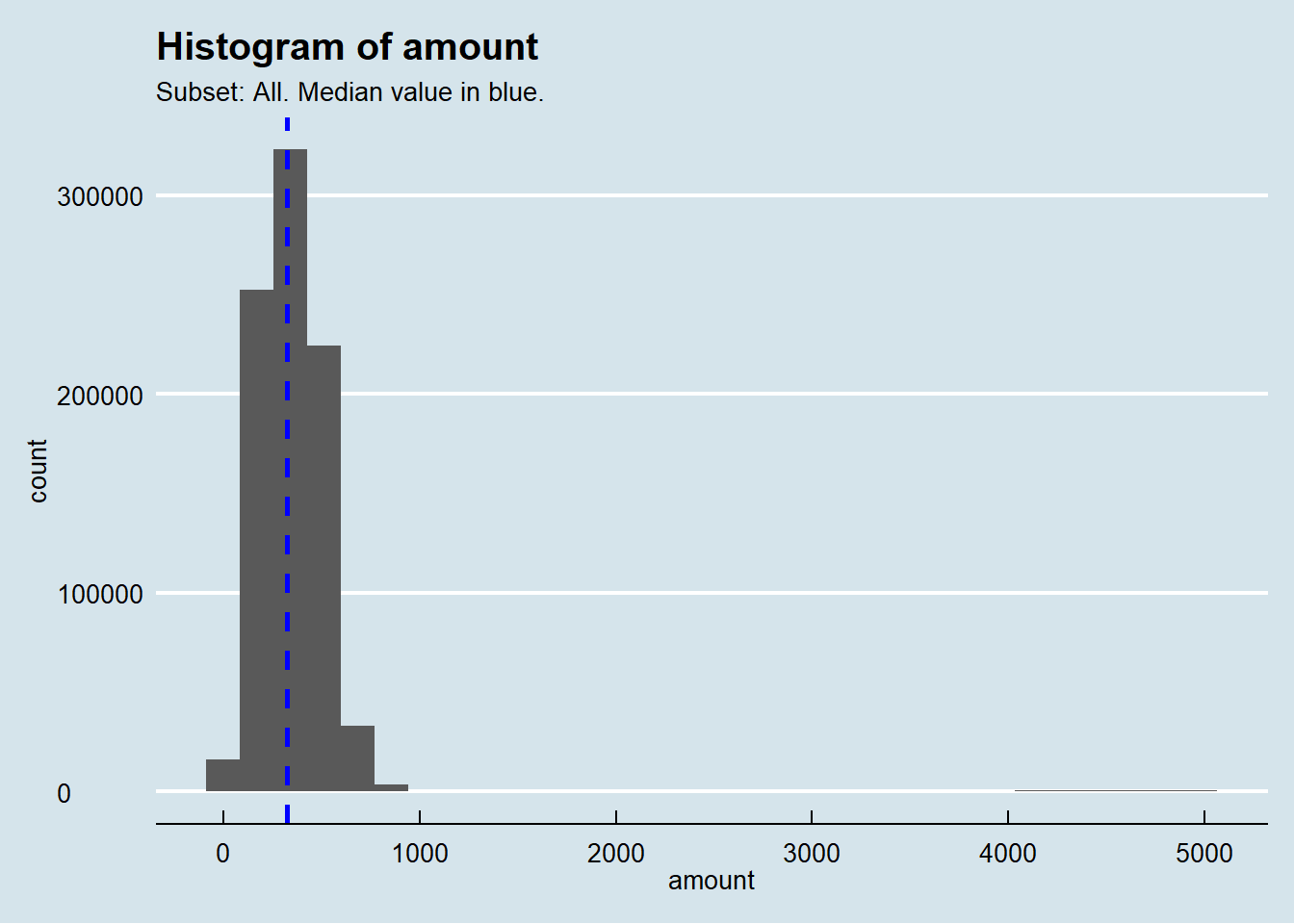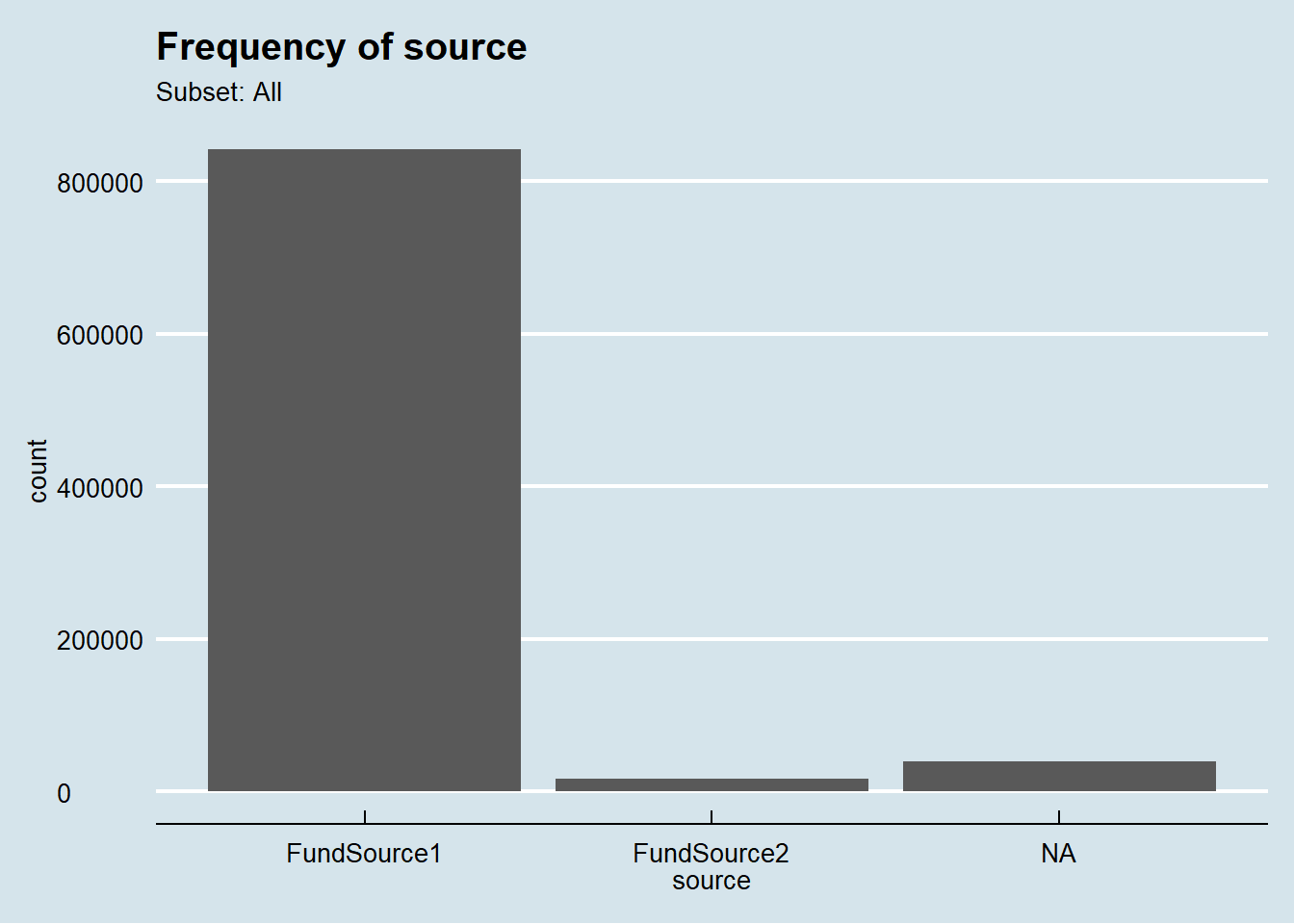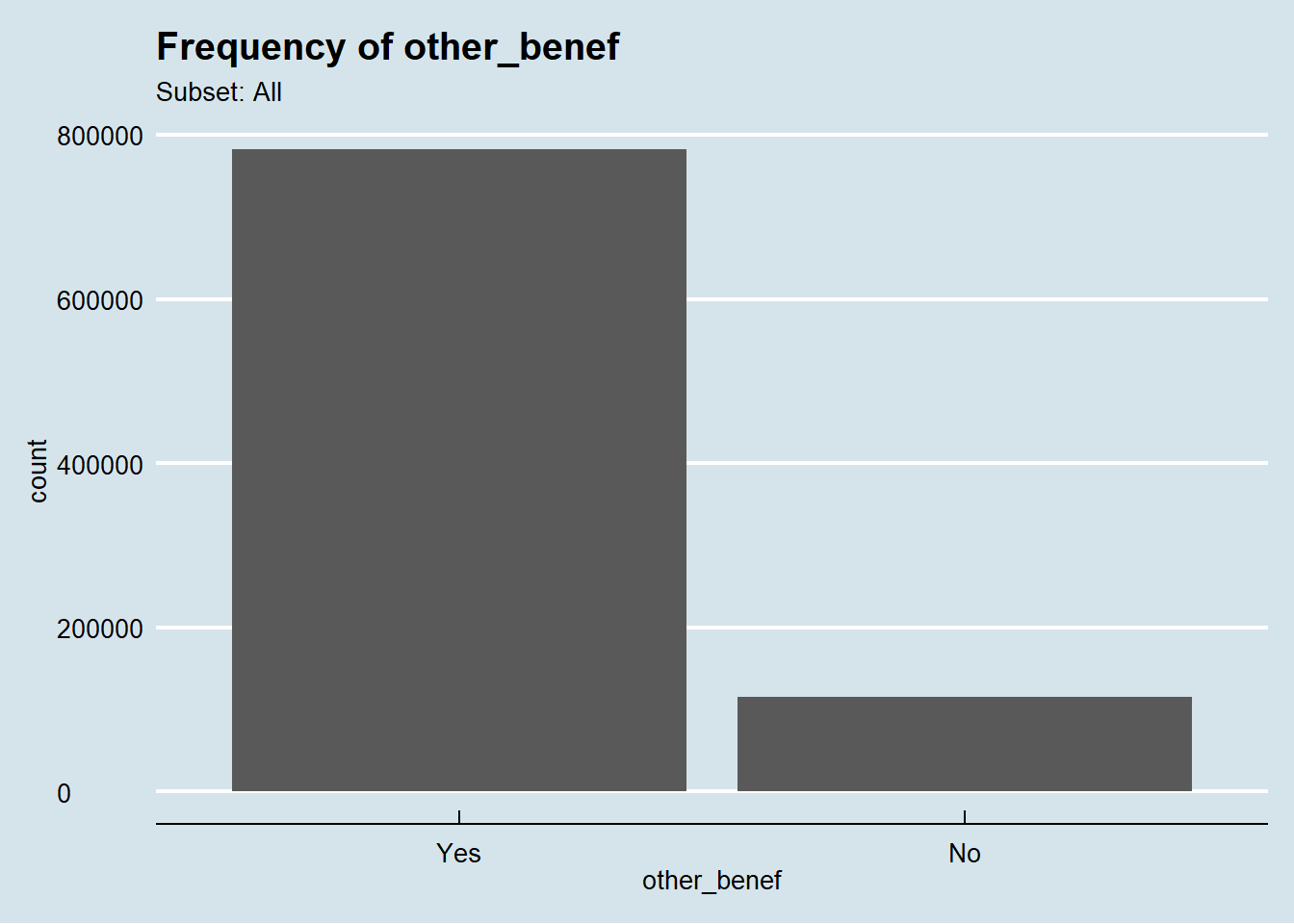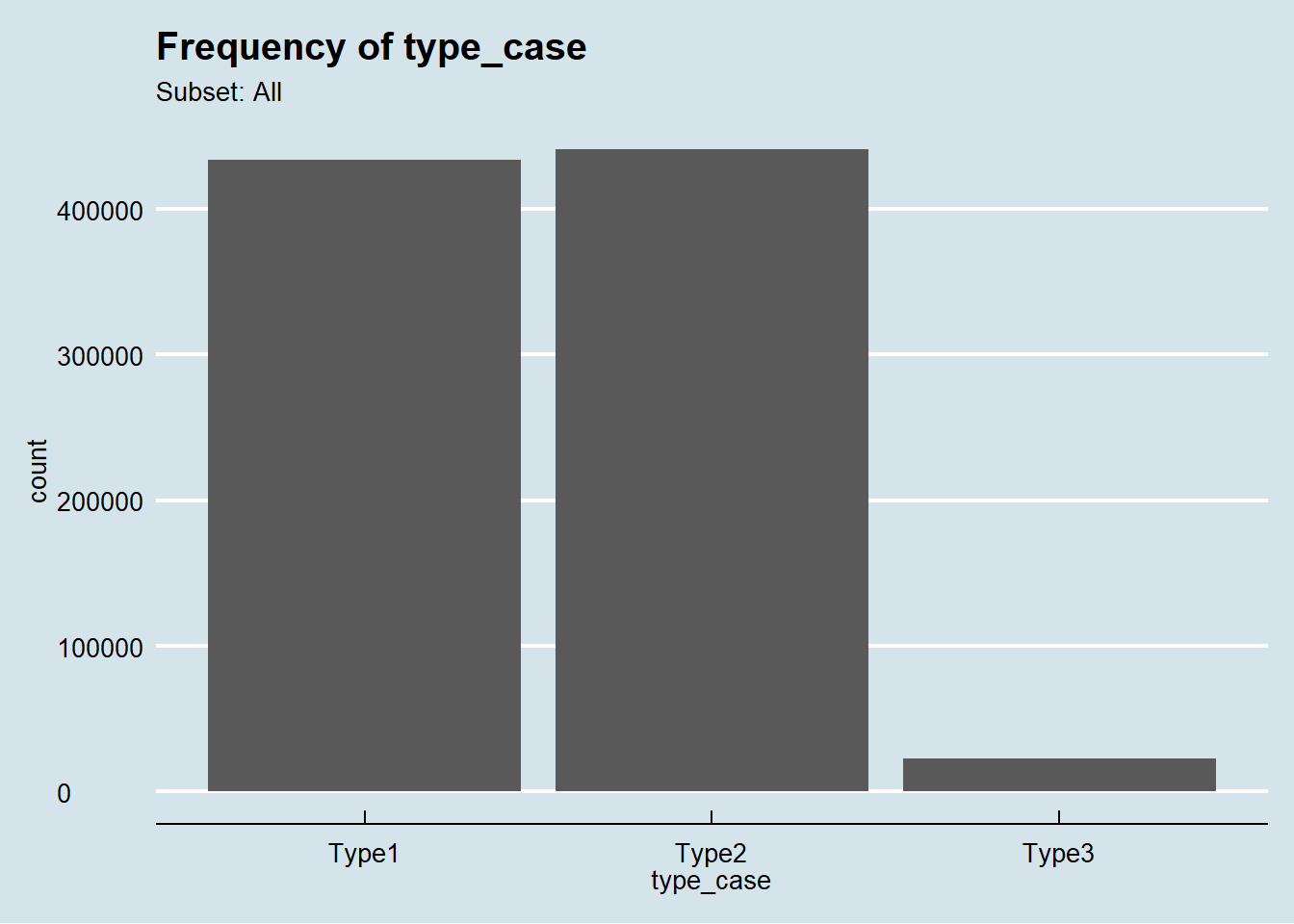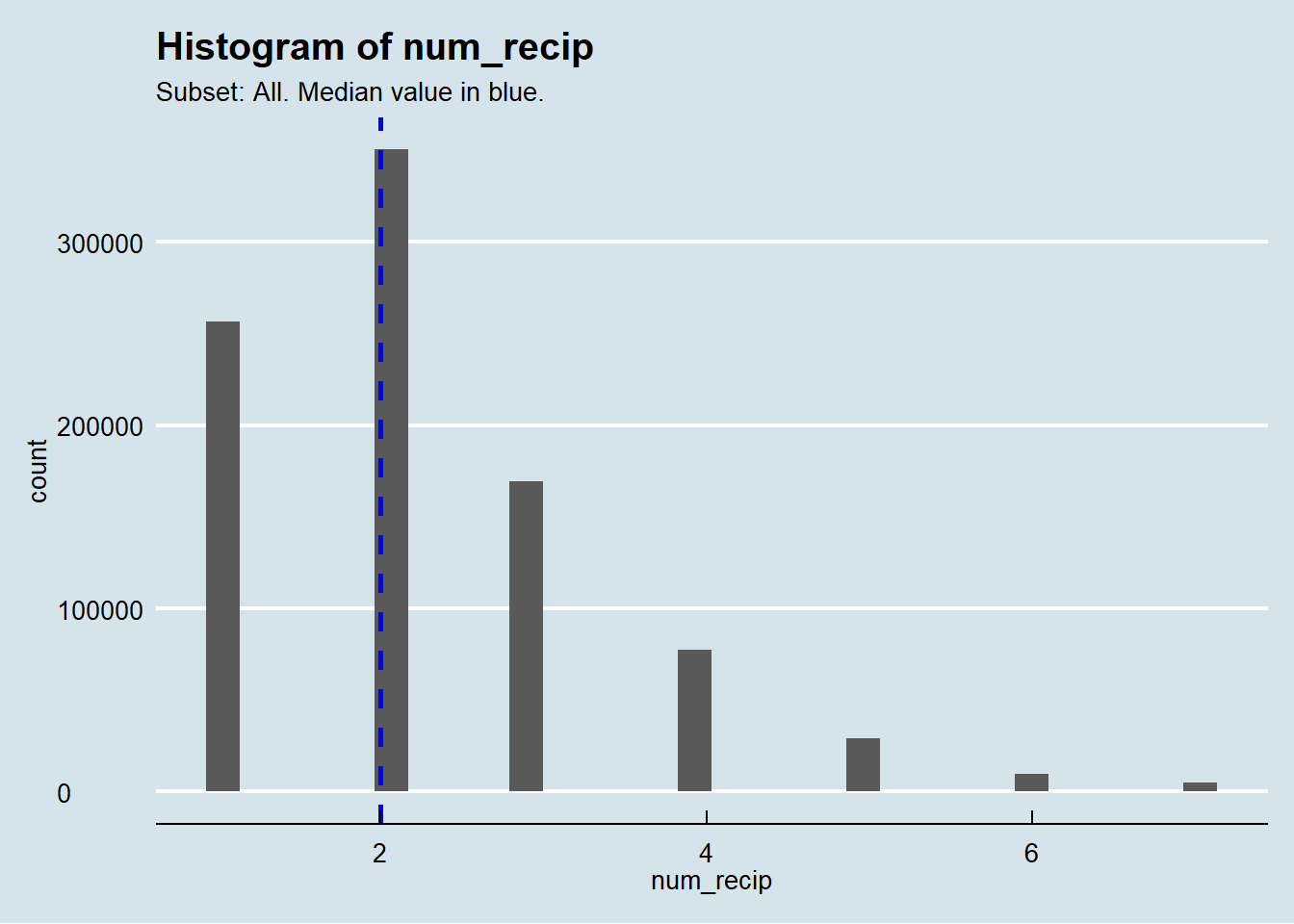Interpretation: When looking at each histogram, consider the following questions about the data:
* One or many peaks?: The peaks are the tallest bars and indicate the most frequent values in the data. Distributions with more than one peak usually indicate that there is more than one group represented in the data. For example, the distribution of height of a population may exhibit two peaks, one representing the most common value for men and the other representing this value for women.
* Should the data be symmetrical? Is it skewed?: Variables such as height, weight, and intelligence measures follow an approximate symmetrical distribution with most values clustered symmetrically near the median and few values on the tails. In skewed distributions, we do not observe such symmetry and it’s common to have one tail of the distribution considerably longer than the other tail. This is typically the case for income data, where there are few people/households with very high income, that makes the tail of extreme values extend longer towards the positive, or right side. If not symmetrical, should the data be skewed to the right? Or to the left?
* Should there be upper or lower limits on data values?: Some variables have natural limits on the possible values of the data. For example, age cannot be negative or larger than, say 120 years; and the profit margin of a company cannot be larger than 100%. Data can also be restricted in the values it can take based on the metadata, such as a variable used to register the number of people living in a household that does not accept more than two-digit numbers. Are these limits apparent in the histogram above? Values beyond these limits could be the effect of errors in data processing or data collection.
* How likely are extreme values of data?: When extreme values are likely, we will observe fatter tails. On the other hand, it is possible to have all data values in a range equally likely (uniform distribution). An example is the distribution of day or month of birth, where we should observe a similar proportion of people in each day or month.

## 6.4 1. Are there units (records) that are missing or not available for the analysis?

Reference Script: completeness\Completeness_unit.Rmd

Description: Ideally, to check that a data file is complete with all expected units (records) included, the units in a file can be compared to a reference list or register. Often, such a register is not available. However, examining whether expected geographic areas are included in the data can help toward assessing completeness of the data file. For example, if data from a particular state is missing from a dataset that should include information from all states, a researcher should investigate why. In a similar manner, it is possible to compare the number of units available for analysis from one time to another to assess whether or not there are missing units for some particular time periods.

### 6.4.1 Completeness over time

To study the completeness over time the user should select the time variable. By default, this program will choose the first time variable specified previously by the user when there is more than one time variable.

Select time variable:

``````##### SELECT TIME VARIABLE #####
time_var <- time_vars
################################

# Check that time variable is not null, that belongs to the time variables set,
# and that it is just one variable.
if (is.null(time_var) |
!all(is.element(time_var, c(time_vars))) |
!(length(time_var)==1) )  {
print("Time variable is not a variable of the time set, or there is more than one time variable")
time_var <- NULL
} ``````

Frequency bar plots:

``````# Frequency bar plots
if (!is.null(time_var)) {
time_units <- nlevels(as.factor(subsetted[[time_var]]))

# To appropriately  label the time axis, we calculate the number of time
# periods to be graphed. If the number exceeds 15, then we use labels
# every 5 time units.
if (time_units >= 15) {
print(ggplot(data=subsetted, aes_string(x = as.factor(subsetted[[time_var]]) )) +
geom_bar() +
theme_economist() +
theme(axis.text.x = element_text(angle = 90, hjust = 1)) +
scale_x_discrete( breaks=function(x) x[seq(1, length(x), by=5)] ) +
labs(x = "Time", y = "Number of units") +
ggtitle(paste0("Frequency by ", time_var), subtitle = paste0("Subset: ",subset_text )) )
} else {
print(ggplot(data=subsetted, aes_string(x = as.factor(subsetted[[time_var]]) )) +             geom_bar() +
theme_economist() +
theme(axis.text.x = element_text(angle = 90, hjust = 1)) +
labs(x = "Time", y = "Number of units") +
ggtitle(paste0("Number of cases by ", time_var), subtitle = paste0("Subset: ",subset_text )) )
}
} else {
print("No valid time variable")
}``````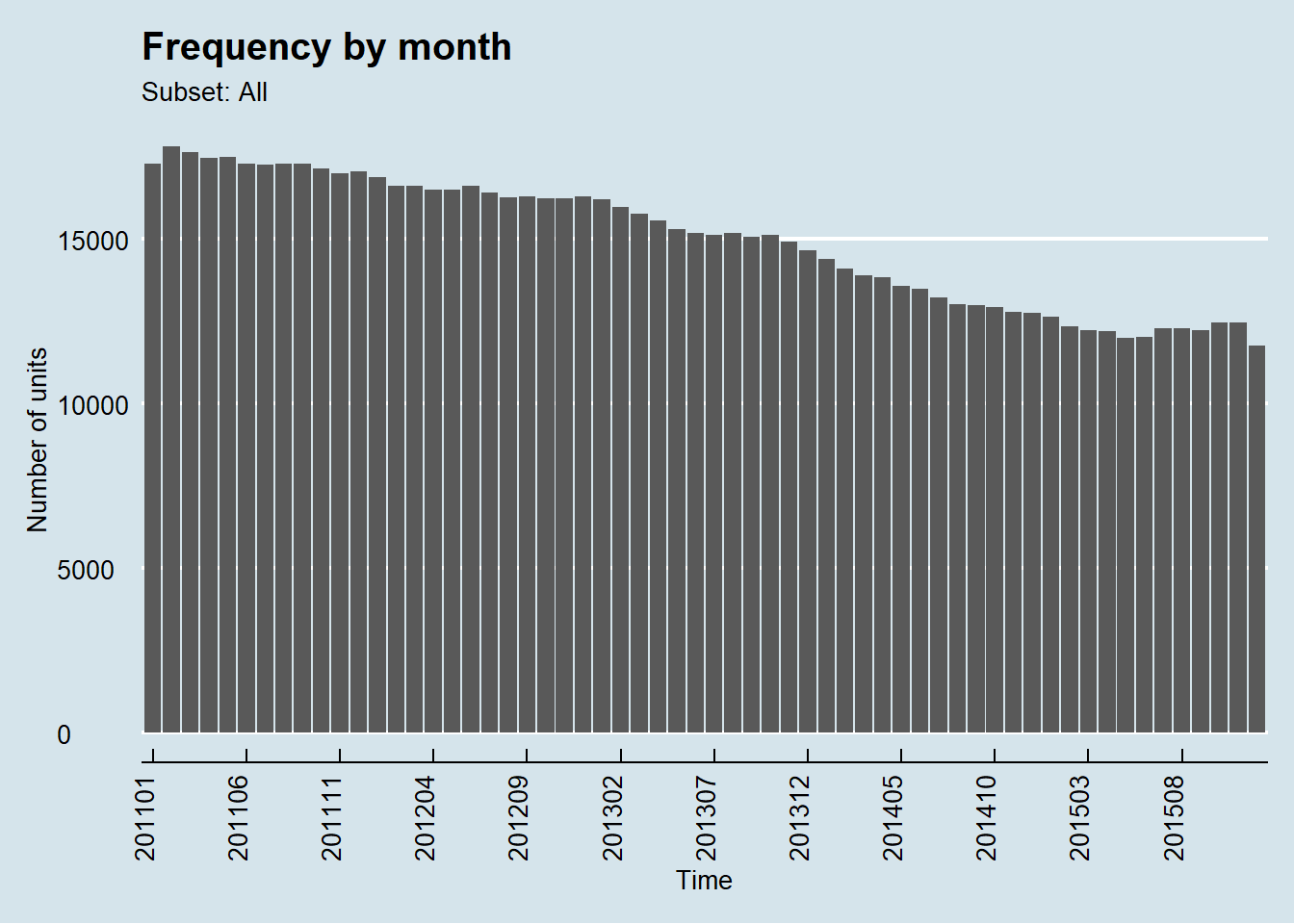Interpretation: The graph shows the trend of the number of units over time. Check if there are discrete jumps in the number of units from one time unit to another. Drastic changes can reflect changes in policy or law, but also problems in the collection of the data.

### 6.4.2 Completeness by geography

To study the completeness by geography the user may select the location variable. The analysis will be run over the same subset of cases as the previous one.

Select location variable:

``````##### SELECT LOCATION VARIABLE #####
location_var <- location_vars
####################################

# Check that time variable is not null, that belongs to the time variables set,
# and that it is just one variable.
if (is.null(location_var) |
!all(is.element(location_var, c(location_vars))) |
!(length(location_var)==1) )  {
print("Location variable is not a variable of the location set, or there is more than one location variable")
location_var <- NULL
} ``````
``##  "Location variable is not a variable of the location set, or there is more than one location variable"``

Frequency bar plots:

``````if (!is.null(location_var)) {
# Frequency bar plots
location_units <- nlevels(as.factor(subsetted[[location_var]]))

print(ggplot(data=subsetted, aes_string(x = as.factor(subsetted[[location_var]]) )) +                   geom_bar() +
theme_economist() +
theme(axis.text.x = element_text(angle = 90, hjust = 1)) +
labs(x = "Geographic identifier", y = "Number of units") +
ggtitle(paste0("Number of cases by ", location_var), subtitle = paste0("Subset: ",subset_text )) )

kable(table(subsetted[[location_var]]), col.names=c(location_var,"Counts"))
} else {
print("No valid location variable")
}``````
``##  "No valid location variable"``

Interpretation: Refer to a list of geographic units expected for the data set, and see if there are any missing for which data would be expected.

## 6.5 Item nonresponse: Examining missing data for variables within records

Reference Script: completeness\Completeness_values.Rmd

Description: These analyses examine the absence of values within the records included in the data file. This section describes the dataset both in terms of the missingness within each variable and also in terms of units with at least one missing value for any analyzed variable. For longitudinal analysis, units with missing values during the analyzed time period are also identified.

A future analysis planned for the toolkit will analyze the characteristics of records with missing data. If certain types of records are more likely to have missing values, analyses may lead to incorrect inferences.

### 6.5.1 Examining proportions of missing values

We plot two graphs in this analysis. The first one shows the proportion of missing values for each variable. The second one shows the number of times (y-axis) that different combinations of variables (x-axis) are missing. The cells shaded in red indicate the variables missing for that specific combination.

Bar plots and missing value pattern matrix:

``````# Bar plots and missing value pattern matrix:
subsetted_aggr = aggr(subset(subsetted,select=c(key_vars, domain_vars, id_vars, time_vars, location_vars)), numbers=TRUE, prop=c(TRUE,FALSE), cex.axis=.7, gap=3, ylab=c("Proportion of missingness","Missingness Pattern"))``````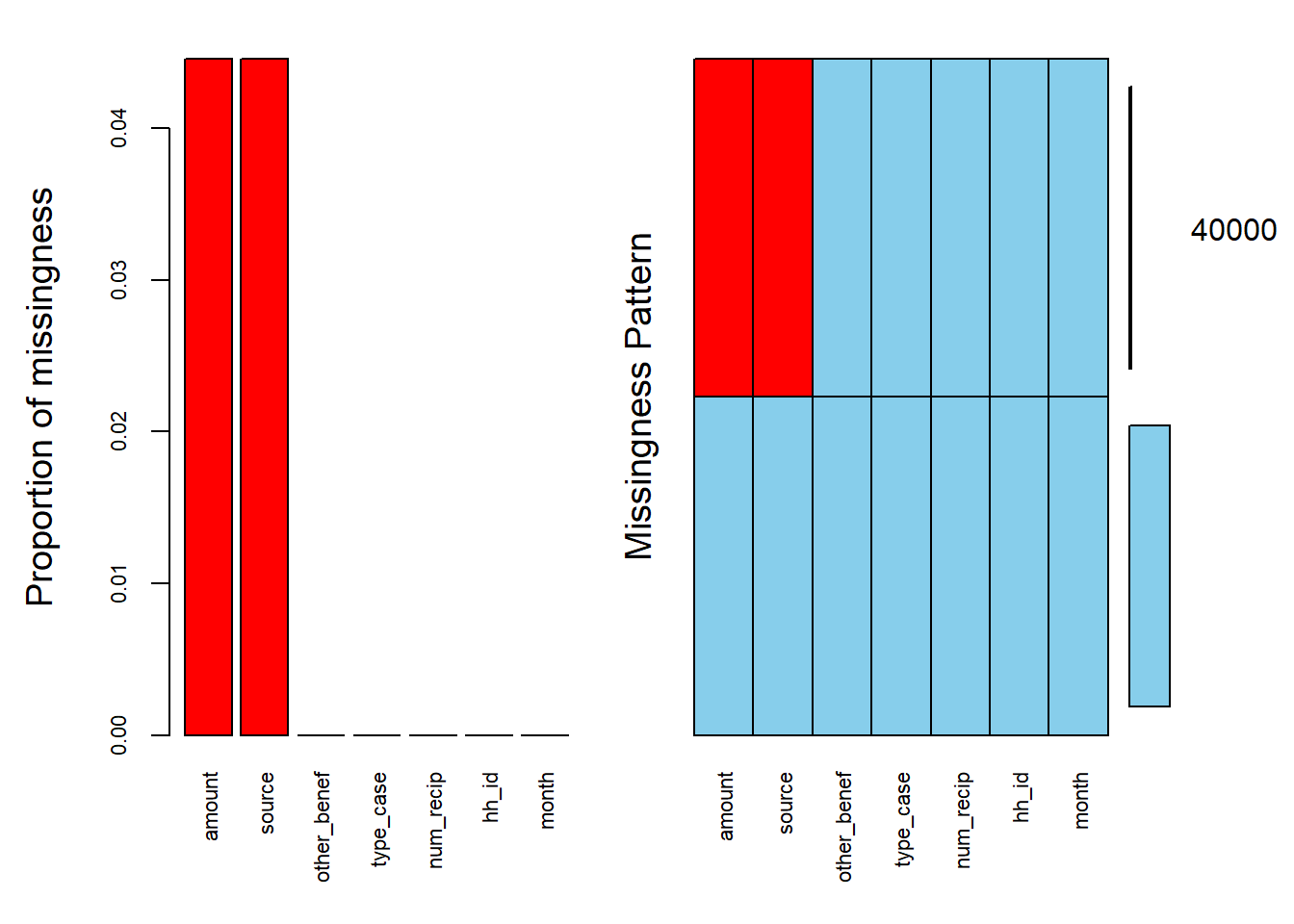Interpretation: The graph on the left shows the proportion of missing values for each variable. Check if the missing values are concentrated in some variables and if these are key or domain variables.

In some cases, it is possible that certain variables are missing together. For example, cases which have the funding source missing may also have a missing value for the amount received. The graph on the right shows whether missing values are concentrated in one variable or in certain combinations of variables. Understanding this missing data structure will inform how analyses may be impacted. Further resources on analyzing data with missing values and addressing missing data using imputation approaches are available from Schafer (1997) and van Buuren (2018).

### 6.5.2 Units with at least one missing value

Here we graph the proportion of units with at least one missing value over time.

``````subsetted\$nmiss <- rowSums(is.na(subsetted)) #>=1
subsetted\$nmiss[subsetted\$nmiss>=1] <- 1
subsetted\$nmiss <- as.factor(subsetted\$nmiss)

# Frequency bar plots
time_units <- nlevels(as.factor(subsetted[[time_vars]]))

if (time_units >= 15) {
print(ggplot(data=subsetted, aes_string(x = as.factor(subsetted[[time_vars]]), fill=subsetted\$nmiss )) +
geom_bar(position="fill") +
theme_economist() +
scale_y_continuous(labels = scales::percent_format()) +
theme(axis.text.x = element_text(angle = 90, hjust = 1)) +
scale_x_discrete( breaks=function(x) x[seq(1, length(x), by=5)] ) +
labs(x = "Time", y = "Proportion of units", fill="Missing") +
ggtitle(paste0("Prop. units with at least one missing value", ""), subtitle = paste0("Subset: ",subset_text )) )
} else {
print(ggplot(data=subsetted, aes_string(x = as.factor(subsetted[[time_vars]]), fill=subsetted\$nmiss  )) +
geom_bar(position="fill") +
theme_economist() +
scale_y_continuous(labels = scales::percent_format()) +
theme(axis.text.x = element_text(angle = 90, hjust = 1)) +
labs(x = "Time", y = "Proportion of units", fill="Missing") +
ggtitle(paste0("Prop. units with at least one missing value",""), subtitle = paste0("Subset: ",subset_text )) )
}``````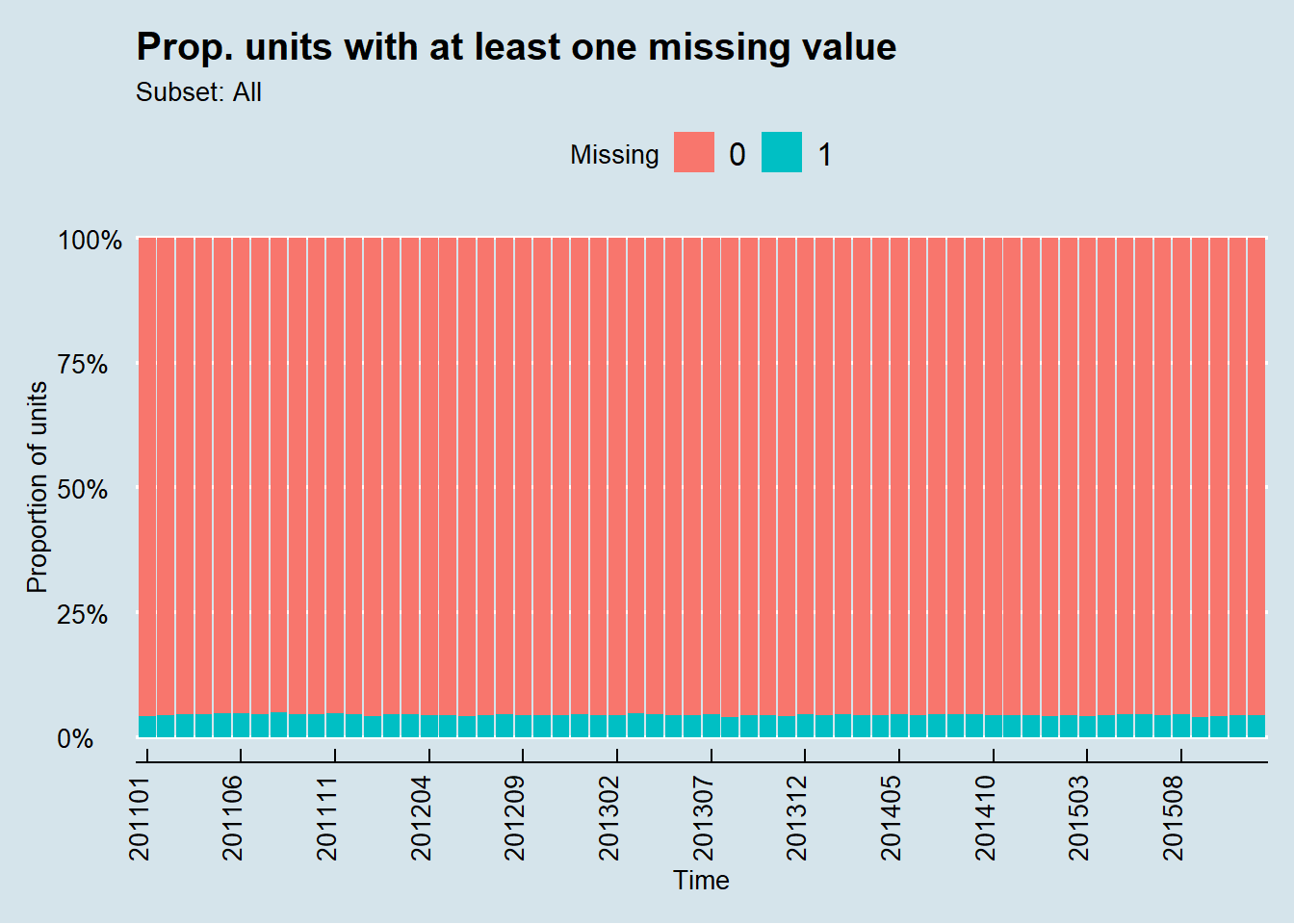Interpretation: Check if units/records with missing values are concentrated in certain months/years. Certain analyses, such as regression models, will typically exclude all records with at least one missing value. Again, imputation is option for addressing missing values, and we refer readers to Schafer (1997) and van Buuren (2018) as resources on imputation.

# 7 Comparability

## 7.1 Are there unexpected patterns in the relationships among variables?

Reference Script: comparability\Comparability_Relationship_among_variables.Rmd

Description: Variables which have a predictable relationship with each other are examined to determine whether that relationship is apparent in the data or not. For example, in some benefit programs, the cash assistance received by the family is expected to be directly proportional to the number of beneficiaries in the household. Additionally, the relationships of other variables are assessed to detect unexpected patterns.

We use tableplots (Tennekes et al., 2011) to help assess if the relationship between two or more variables exists. A tableplot can aid in the exploration of large data files by summarizing it over a set of variables. Each variable is represented in a column, where each row is an aggregate of a given number of units.

For each column, all records are sorted by values of a variable in the table. The variable should be chosen for its helpfulness in assessing the relationship with other variables. The default method partitions the sort variable into percentiles and graphs its approximate distribution by percentile. Thus the y axis presents percentiles of the sort variable.

For continuous variables, the length of the light blue bar represents the mean, and the dark blue bars represent the standard deviation. For discrete variables, different colors indicate the frequency of each category.

We recommend using at least one key variable as the sort variable that the user can specify. By default, this program will choose all numeric key variables and will generate a tableplot sorted by each variable. Additionally, the user can specify subsetting parameters for the data file (in Toolkit.Rmd), such as specific time ranges.

### 7.1.1 Relationships among key variables

To study the relationship between key variables, the user should select the sorting variable among key variables.

Select sorting variable:

``````##### SELECT SORTING VARIABLE #####
sort_var <- NULL
###################################

# If no sorting variable is specified or if sorting variable is not a key variable,
# we choose all the numeric variables as sorting variable.
# If no numeric variables, we choose all non-numeric key variables.
if (is.null(sort_var) | !all(is.element(sort_var, key_vars)) )  {
sort_var <- NULL
for (i in key_vars) {
if (is.numeric(analysis_file[[i]])) {
sort_var <- c(sort_var,i)
}
}
if (length(sort_var) == 0) {
sort_var <- key_vars
}
}``````

Tableplots: We create one tableplot for each sorting variable.

``````# Tableplot with key variables
if (!is.null(sort_var)) {
options(ffmaxbytes = min(getOption("ffmaxbytes"),.Machine\$integer.max * 12))
for (i in sort_var) {
tableplot(subsetted,
select_string = key_vars,
sortCol = i,
scale = "lin",
title = paste0("Distribution of key variables. Sorted by: ",i,"\nSubset: ",subset_text))
}
} else {
print("No valid sorting variable")
}``````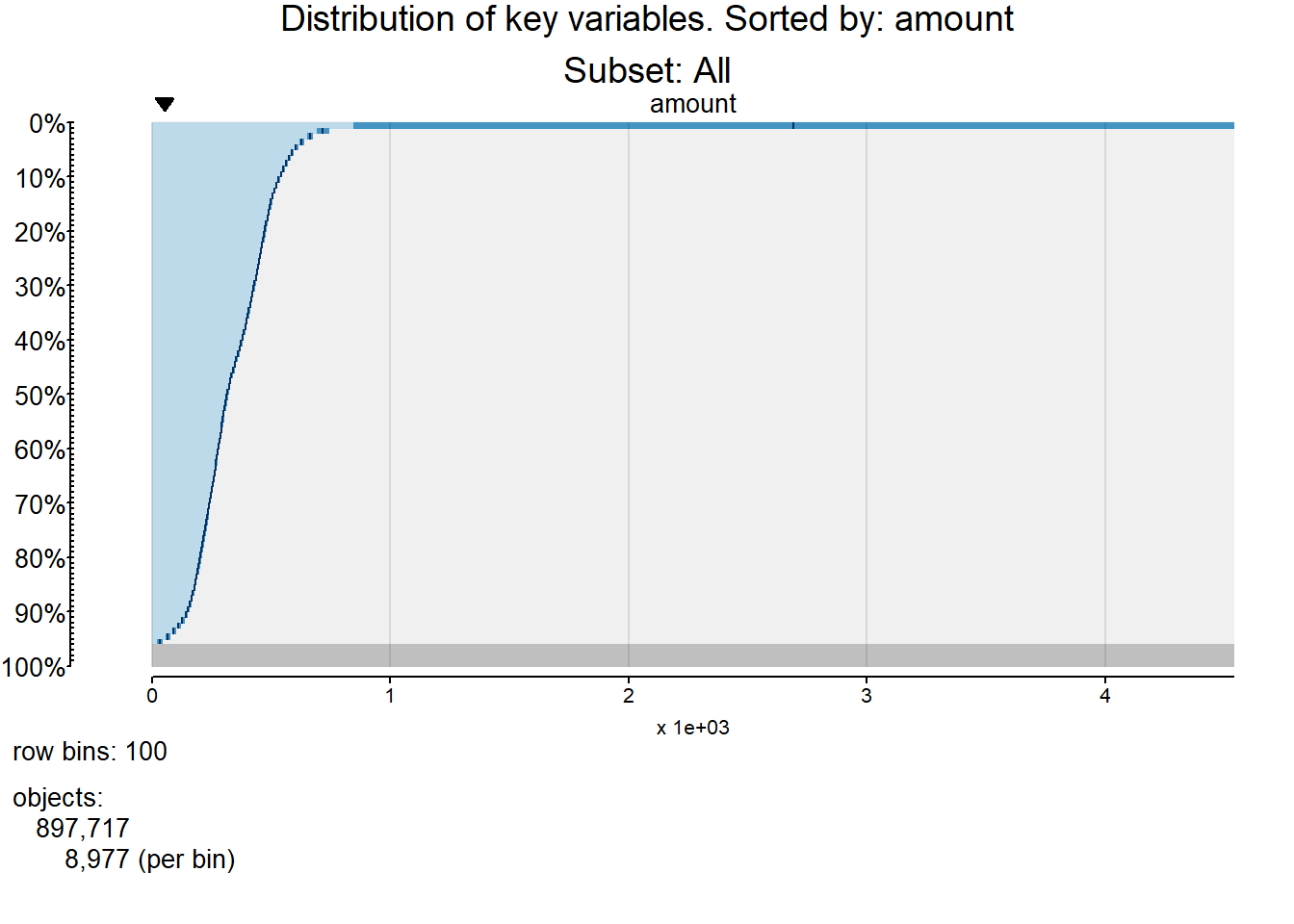What to look for in tableplots:
- Relations between variables: Use the graph to check relationships between variables there are any unexpected trends that warrant further inquiry. - Discontinuities in sorted variables: Among sorted variables, look for jumps in the distribution of continuous variables. Note that discrete variables may exhibit discrete jumps which are perfectly fine, such as increasing or decreasing the number of household members by one or more.
- Discontinuities in unsorted variables: Among unsorted variables, look for discontinuities in continuous and categorical variables, and note the direction of the jump. Some jumps are fine, such as jumps in the cash received by the household when the number of recipients increased. - Range of values: Look at the range of values of each variable to detect variables with particularly high or low range that may cast doubt about the validity of the values.
- High standard deviation or discrete jumps in the standard deviation: Look for high standard deviations, which may indicate problems in the validity of the values. For example, in some cases missing values are codified with values such as 999999 or 0 which will increase the variability of the data. Also, look for drastic changes in the standard deviation.
- Highest and lowest values: Check the highest and lowest values of the sorted variable and how they relate to the other variables.

### 7.1.2 Relationships among key and domain variables

Here we include domain variables to further assess the relationship between key and domain variables. The same sorting variable and subsetting parameters are used.

Tableplots:

``````#Tableplot with key and domain variables
if (!is.null(sort_var)) {
for (i in sort_var) {
tableplot(subsetted,
select_string = c(key_vars, domain_vars),
sortCol = i,
scale = "lin",
title = paste0("Distribution of key/domain variables. Sorted by: ",i,"\nSubset: ",subset_text))
}
} else {
print("No valid sorting variable")
}``````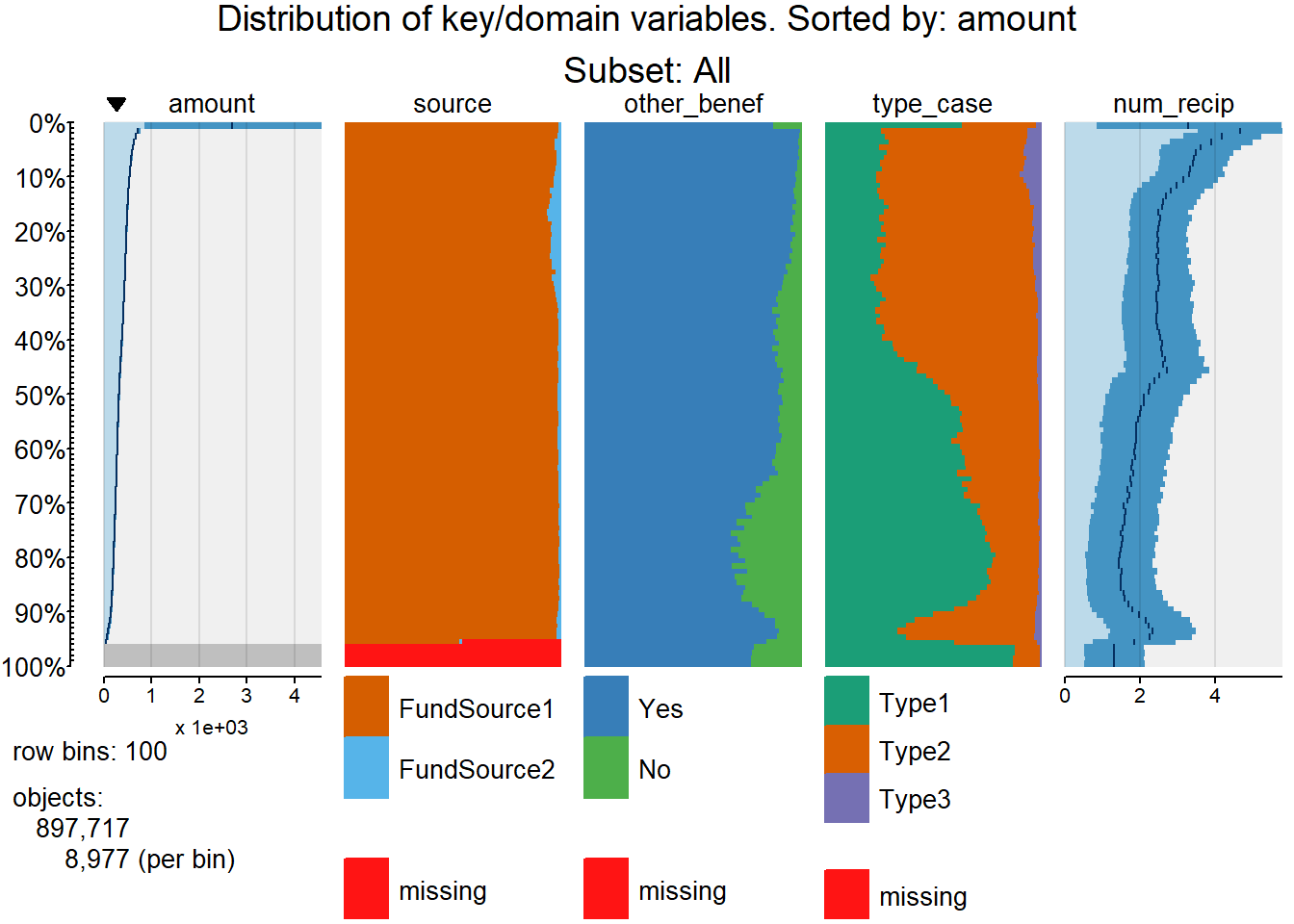Interpretation: In addition to the guidance provided in the previous subsection, check if there are subgroups of units that would be relevant to analyze separately. For example, with administrative data for cash assistance, units with only one recipient may exhibit a different behavior than units with more than one recipient, such as higher average amount received.

### 7.1.3 Relationships among key and domain variables by subgroup

In some cases, it is relevant to analyze the relationship among variables for different categories of units. This is the case when we expect that data will exhibit different patterns in each subgroup. For example, in the case of benefit receipt, cases that only have children as recipients show certain characteristics that make them different from other cases, such as the exemption from federal time limits. In time-series or longitudinal analysis, it is possible to assess the relationship between variables for different time intervals.

The user should select one categorical variable that identifies the different groups that want to be analyzed. The analysis works best when the variable has less than five categories. By default, the system will use the first domain variable. Tableplots will be sorted by one variable that can be defined by the user. In case it is not specified, it will be the first continuous key variable.

Select the subgroup variable:

``````##### SELECT SUBGROUP VARIABLE #####
subgroup = domain_vars
####################################

# If no subgroup variable is specified or if subgroup variable is not a key,
# domain, time, or location variable, or if there is more than one subgroup
# variable, we skip this section.
if (is.null(subgroup) |
!all(is.element(subgroup, c(key_vars, domain_vars, location_vars, time_vars))) |
!(length(subgroup)==1) )  {
print("Subgroup variable is not a key,domain, time, or location variable, or there is more than one subgroup variable")
subgroup <- NULL
} else if (nlevels(as.factor(subsetted[[subgroup]])) >= 15) {
print("Too many levels in the subgroup variable")
subgroup <- NULL
}``````

Tableplots: We graph as many tableplots as categories of the subgroup variable.

``````# Select just the first sorting variable
sort_var <- sort_var

if (!is.null(subgroup) & !is.null(sort_var)) {
for ( i in levels(subsetted[[subgroup]]) ) {
data5 <- subset(subsetted,subsetted[[subgroup]] == i)
if (nrow(data5) > 0) {
tableplot(data5,
select_string = c(key_vars, domain_vars),
sortCol = sort_var,
scale = "lin",
title = paste0("Tableplot sorted by: ",sort_var, ". Where ",subgroup, "= ", i,"\nSubset: ",subset_text))
}
}
} else {
print("No valid sorting or subgroup variable")
}``````